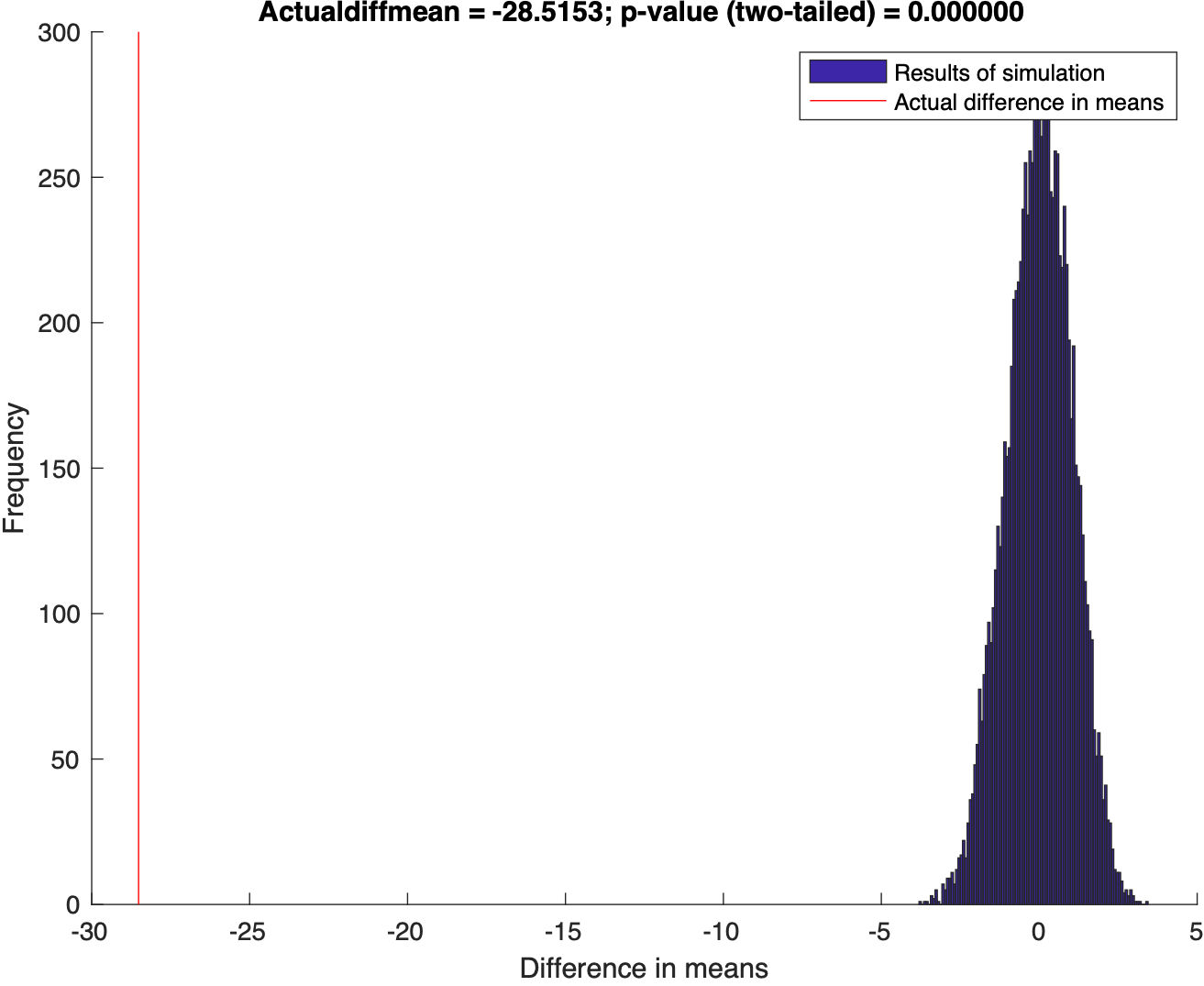## Introduction to Earth Data Analysis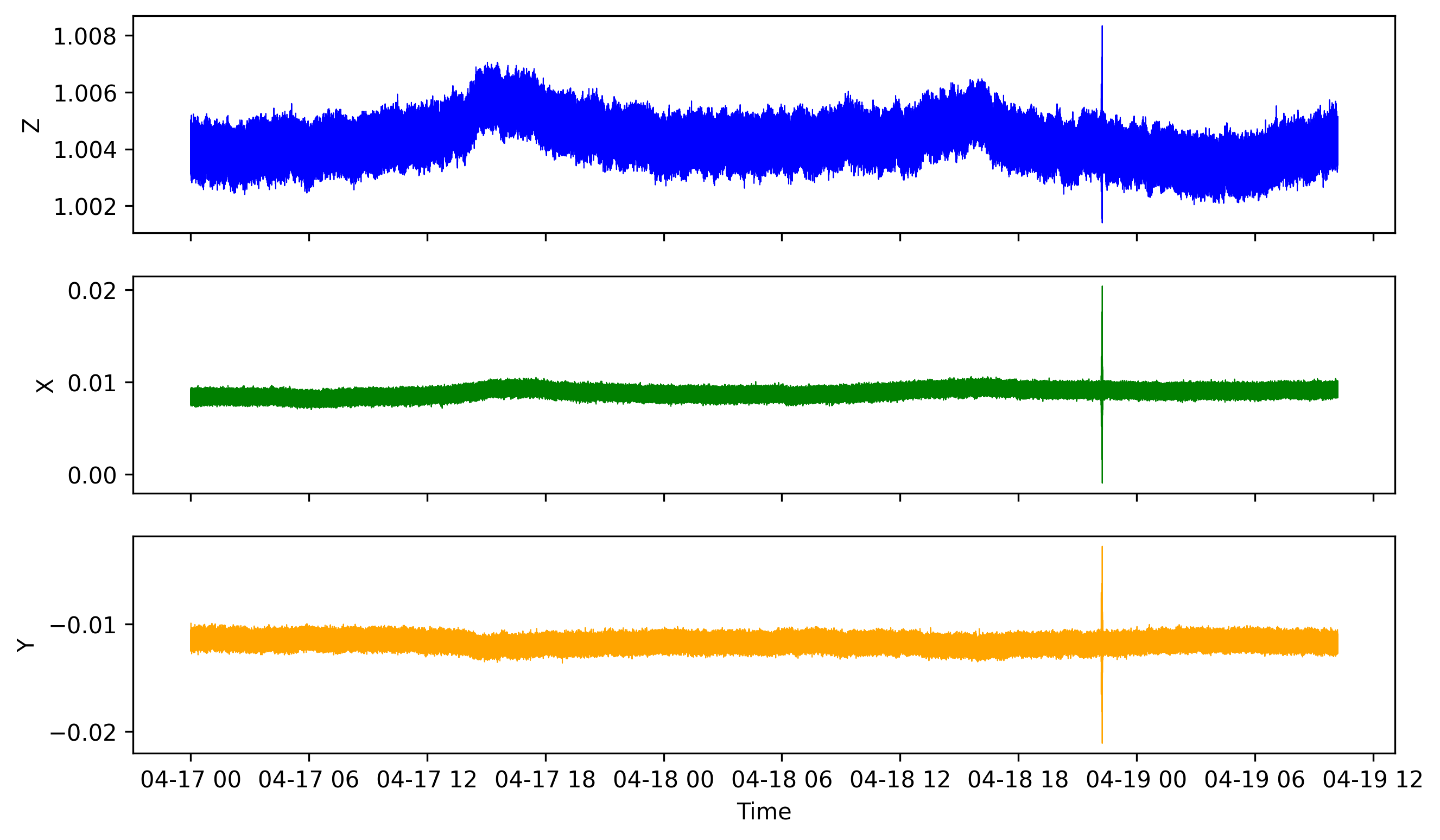## How to analyze a huge data file with pandas (codes included)

We learn how to read huge csv file containing time series data by breaking it into chunks and then visualizing it with matplotlib## How to start using pandas immediately for earth data analysis (codes included)

This tutorial gives a brief description of scientific computing using Pandas by introducing Series, DataFrame, Pandas common operations, methods, conditional...## Introduction to numpy for scientific computing

This tutorial gives a brief description of scientific computing using numpy by introducing arrays, methods, attributes, random numbers, indexing, broadcastin...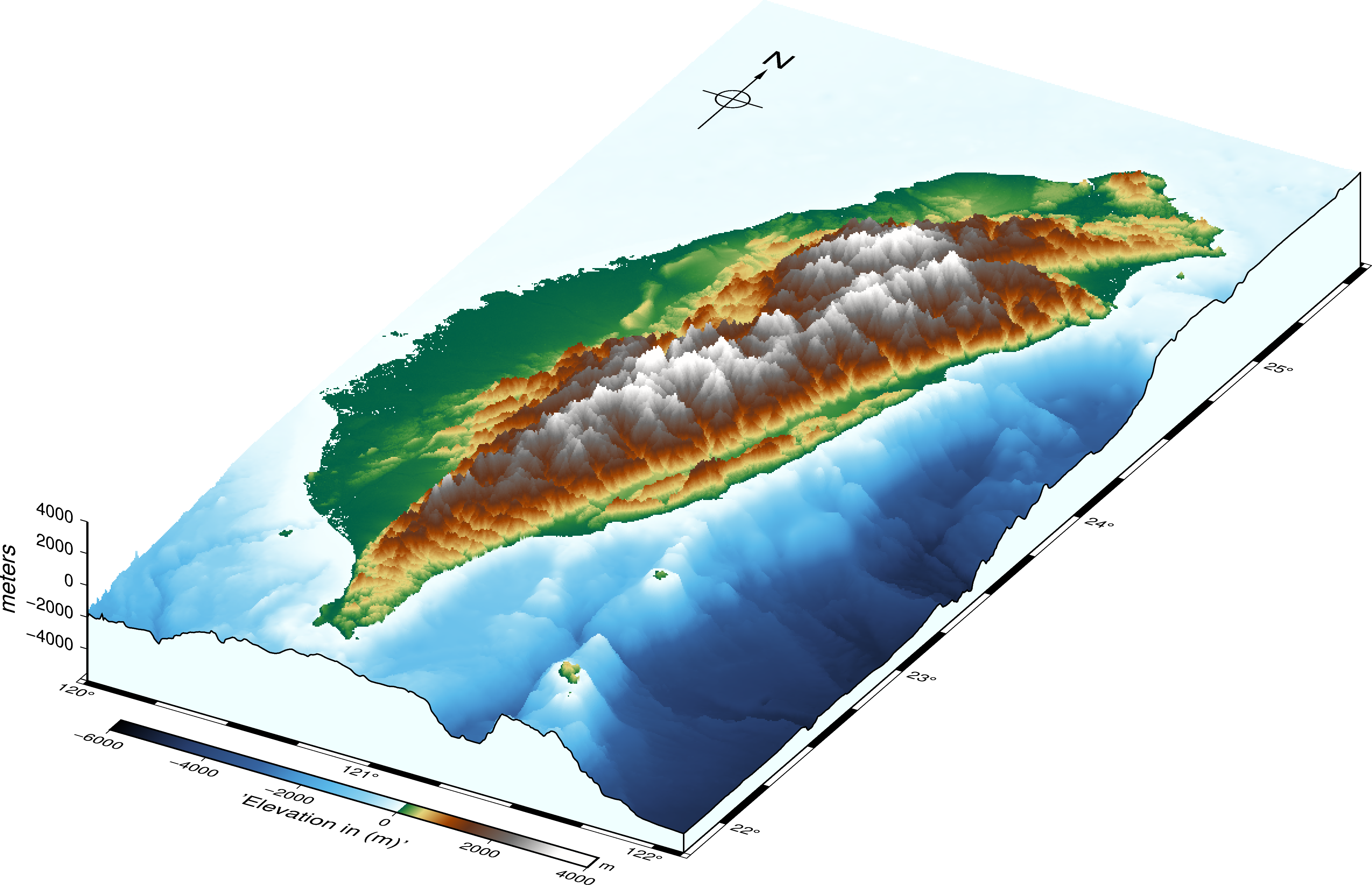## Three-dimensional perspective map of taiwan using gmt and pygmt (codes included)

We learn how to make the three-dimensional map using both GMT and PyGMT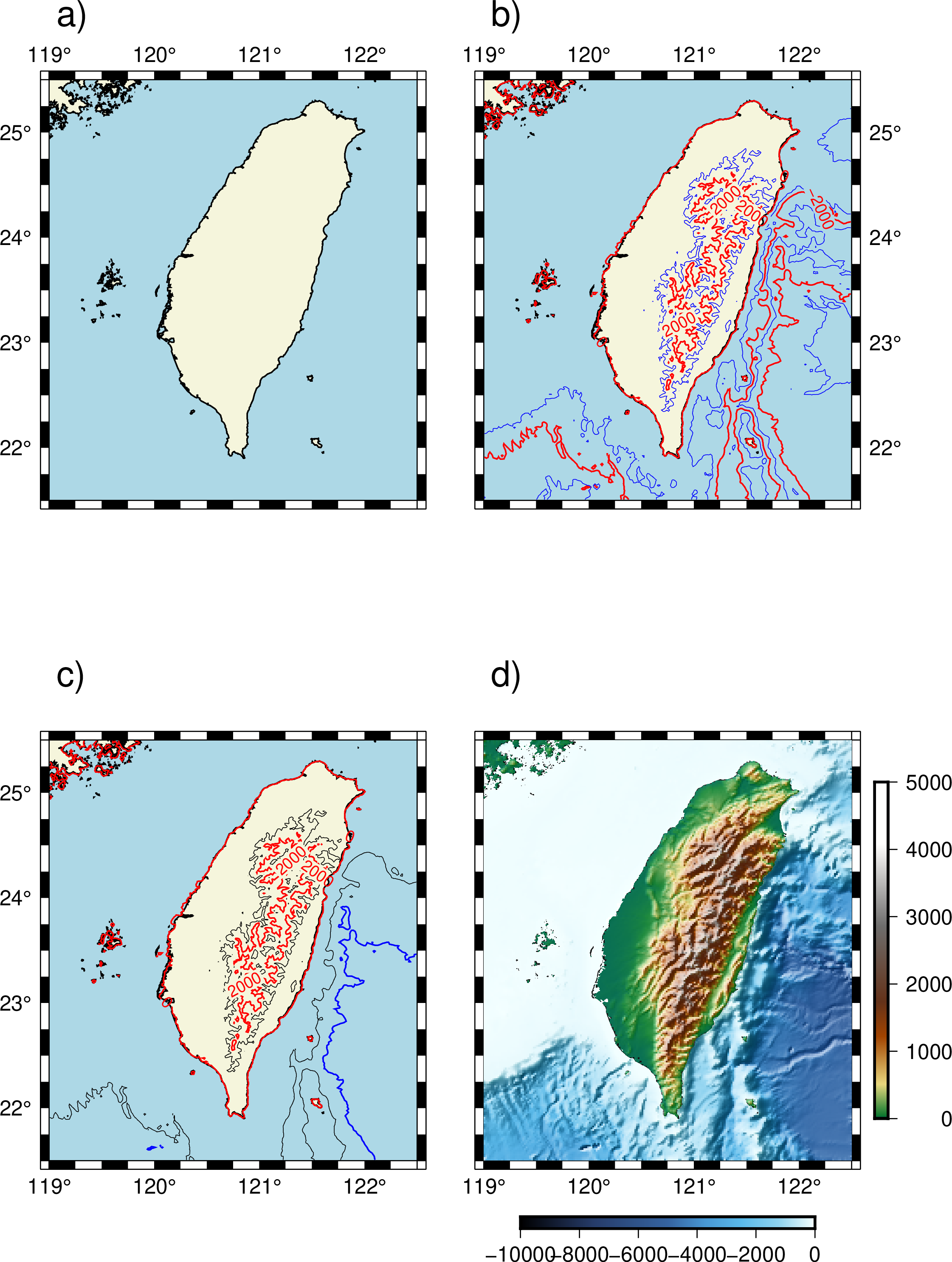## High-quality maps using the modern interface to the generic mapping tools (codes included)

GMT or generic mapping tools have become synonymous with plotting maps in Earth, Ocean, and Planetary sciences. It can be used for processing data, generatin...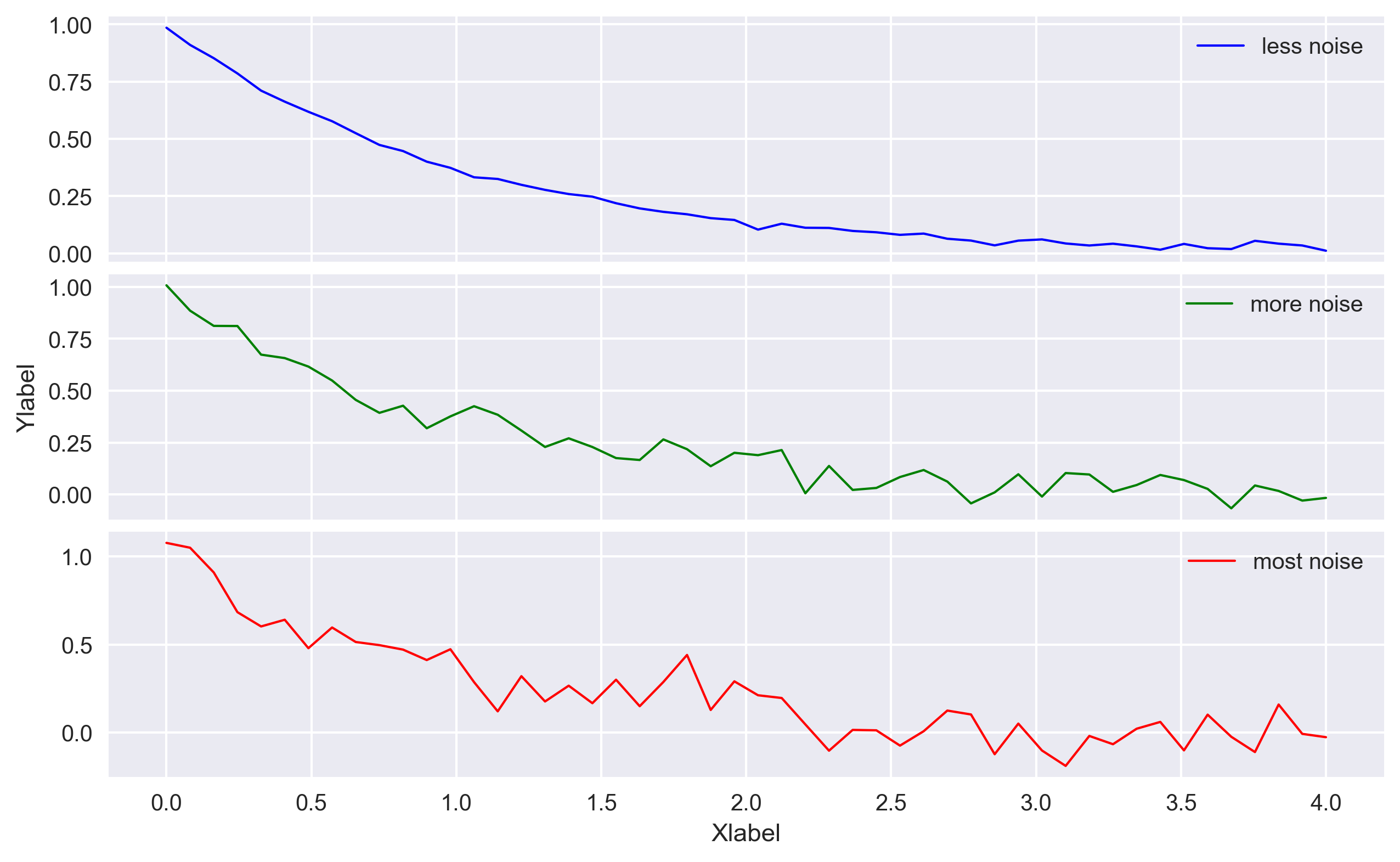## Advanced 2d plots with matplotlib in python (codes included)

Codes for plotting advanced 2D plots using matplotlib library in Python. Includes simple 2D plot, error bars, bar graphs, histograms, multiple plots, etc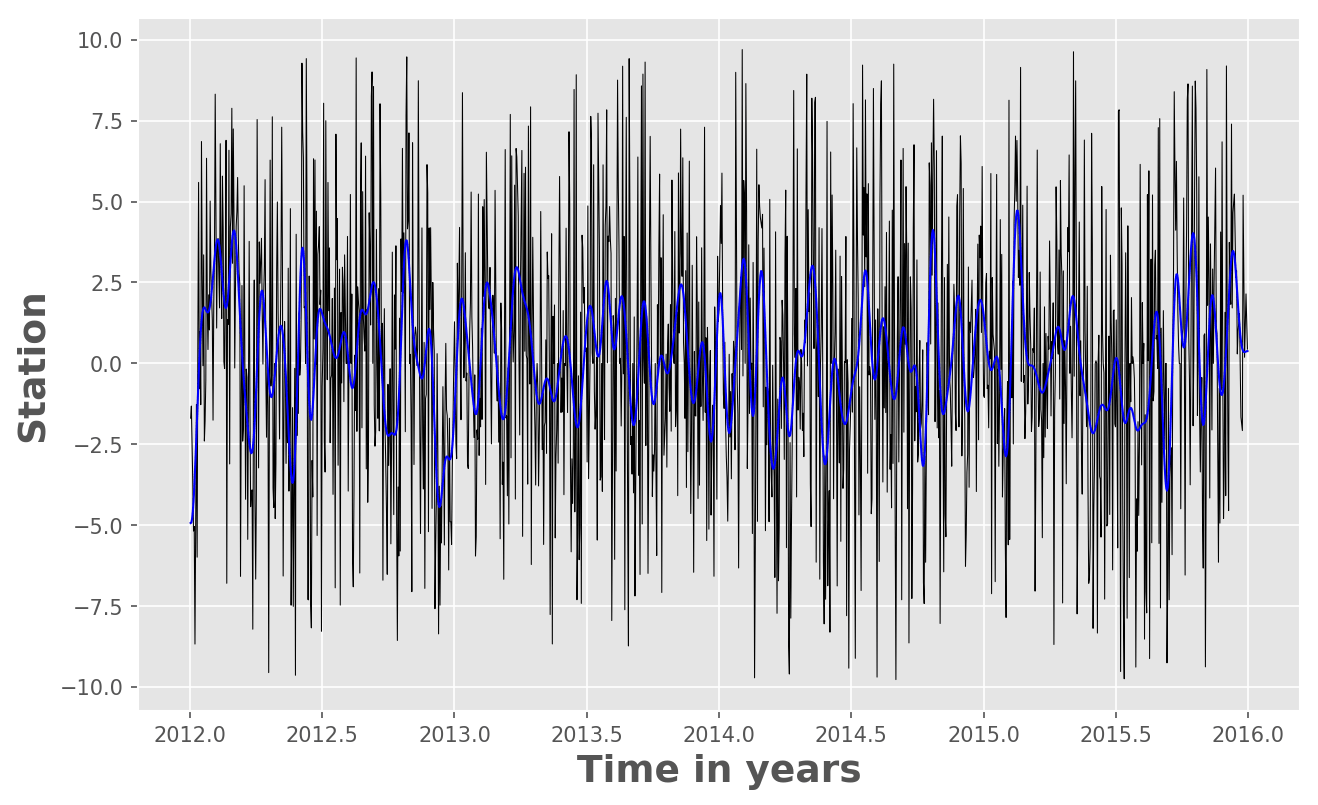## Time series analysis in python: filtering or smoothing data (codes included)

In this post, we will see how we can use Python to low-pass filter the 10 year long daily fluctuations of GPS time series. We need to use the “Scipy” package...## Pygmt: high-resolution topographic map in python (codes included)

A simple tutorial on how to plot high resolution topographic map using GMT tools in Python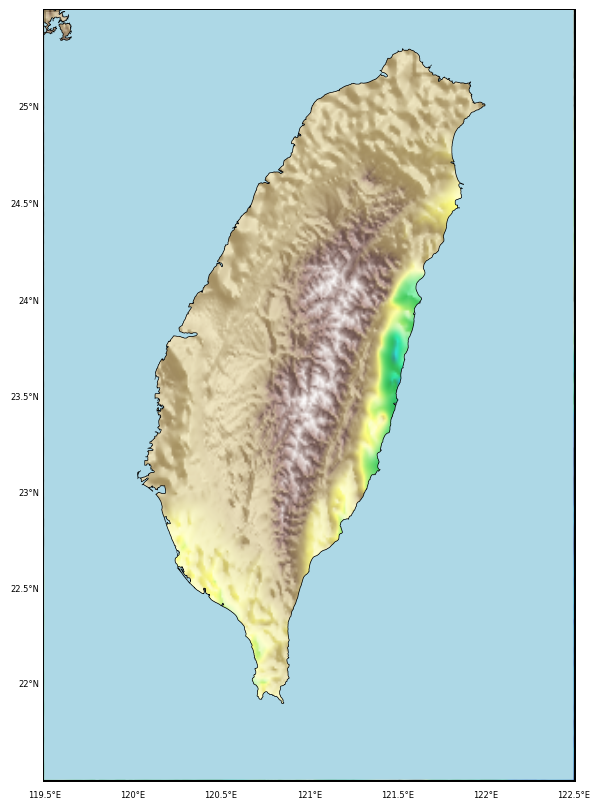## Topographic map clipped by coastlines in python (codes included)

This post demonstrate how to use Python to set up clip topographic map based on coastlines.## Using github for team collaboration

Tutorial on how to use Git and GitHub for team collaboration on a project. Content includes installing, setting up, creating a repository, making commits, un...## Introduction to python for beginners

In this tutorial post, I give a quick demo of how to install Python (using anaconda) and then getting started with writing simple scripts.

## Methods and Algorithms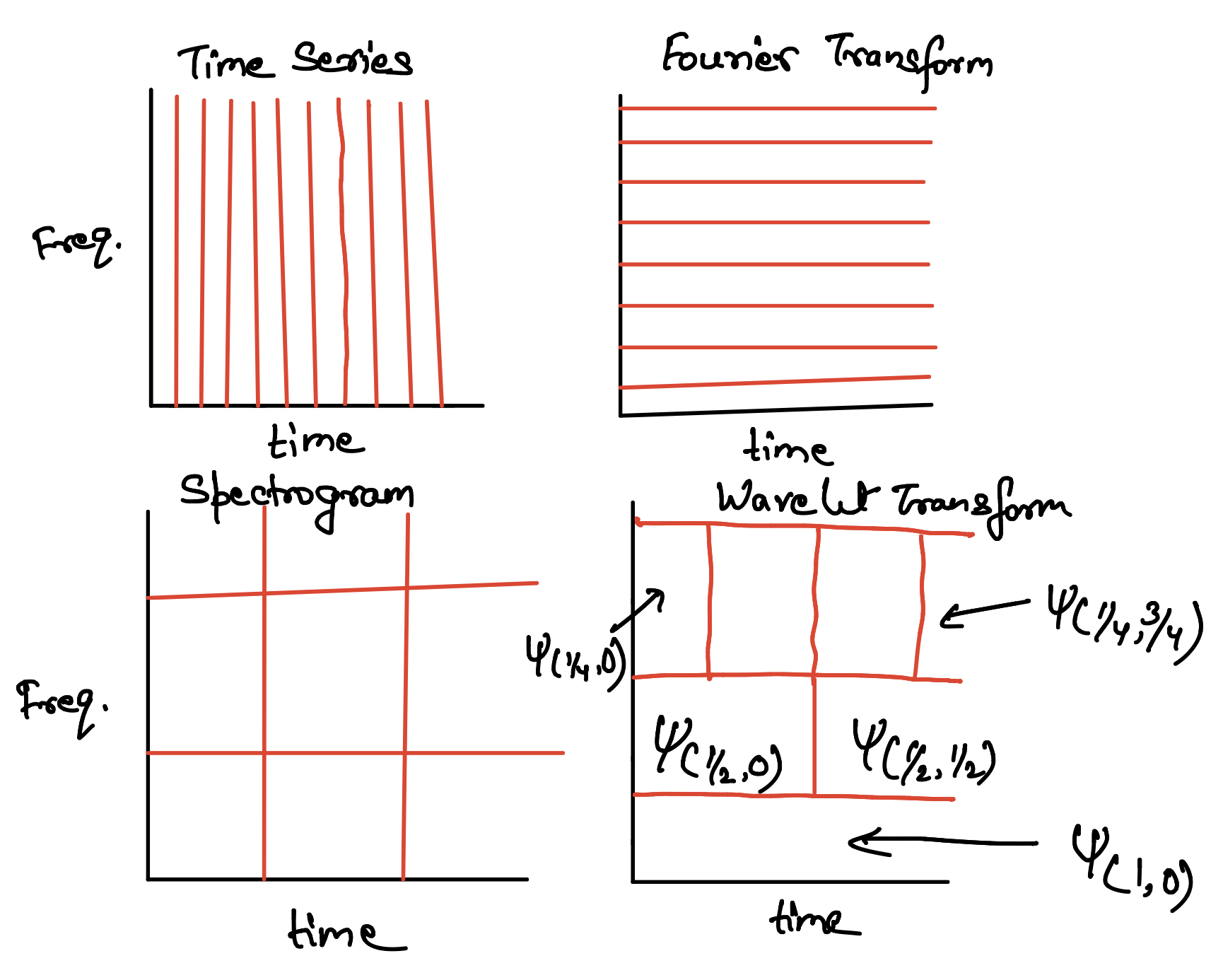## Towards multi-resolution analysis with wavelet transform

We will learn the basic concepts of wavelet tranform and multi-resolution analysis starting from the Fourier Transform, and Gabor Transform.## Wavelet analysis applied to the real dataset in a quick and easy way

An introduction to the wavelet analysis for a real geophysical data set. I compared the analysis to the Fourier analysis. Codes included!## Genetic algorithm: a highly robust inversion scheme for geophysical applications

An introduction to the basics of genetic algorithm along with a simple numerical example and solution of an earthquake location problem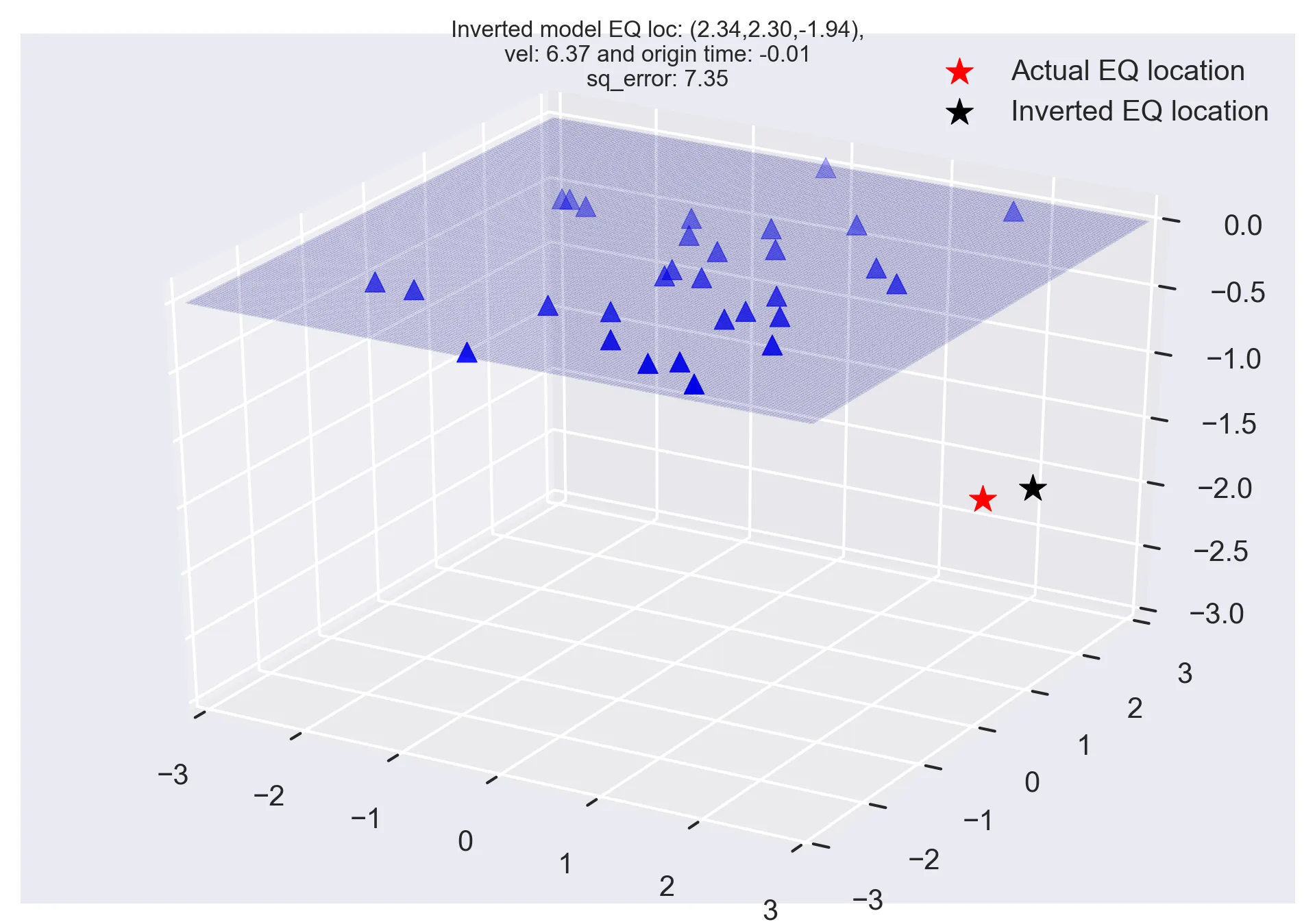## Monte carlo methods and earthquake location problem

The common geophysical problems most often have multimodal objective function with many possible minima. In this post, we will look into the Monte Carlo meth...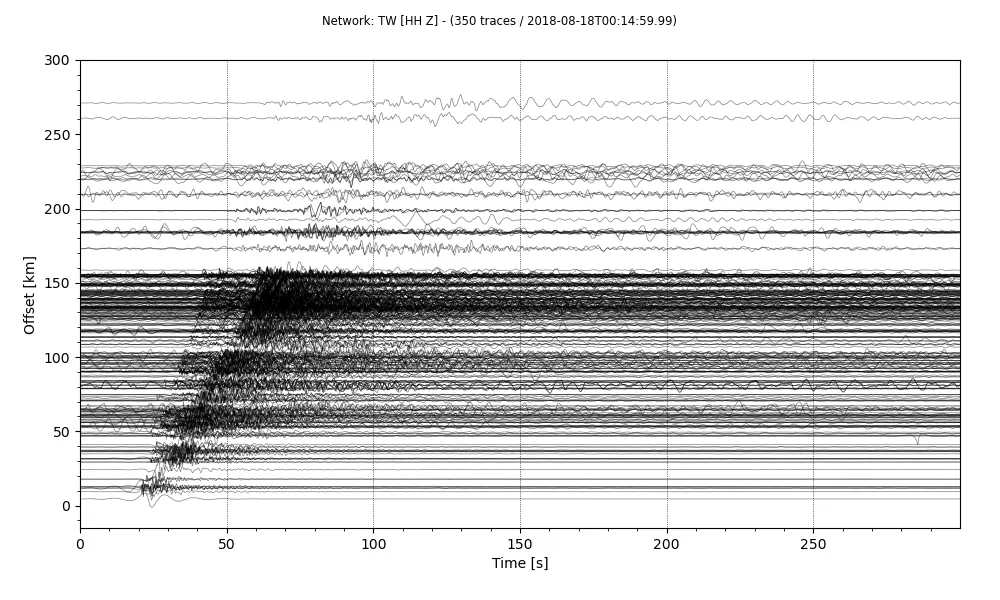## Plotting a record section using obspy

Quickly plot record section of a stream using Obspy. I will introduce you how to make a stream from a set of SAC data, plot the record section and store it a...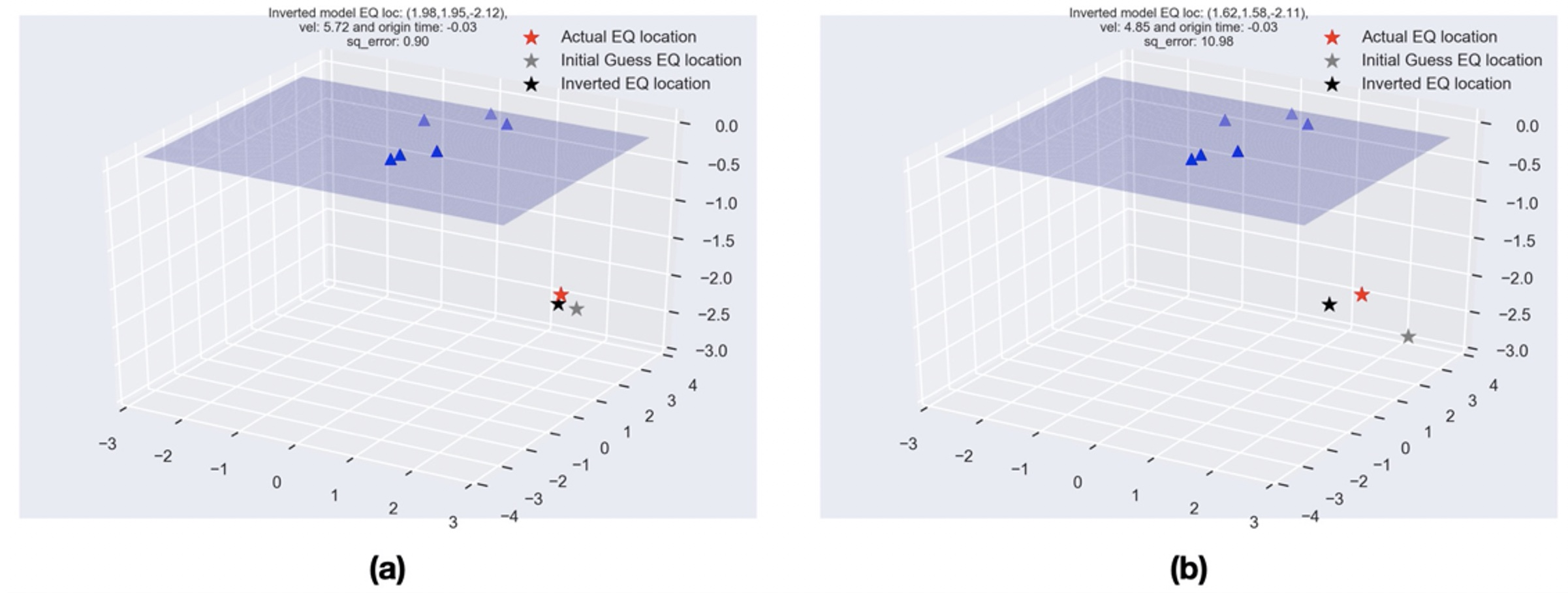## Least-squares method in geosciences

Least-squares method is a popular approach in geophysical inversion to estimate the parameters of a postulated Earth model from given observations. This meth...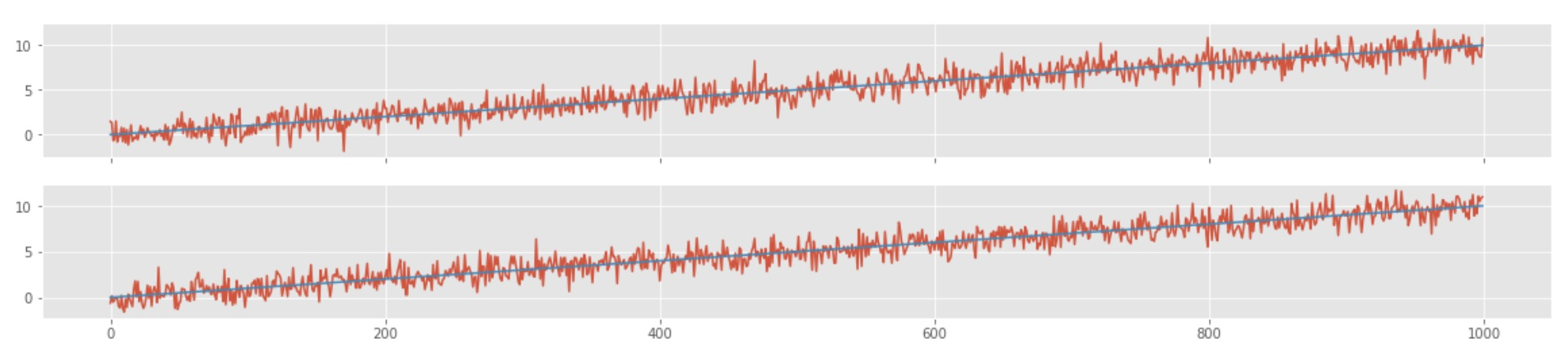## How to avoid common mistakes in analyzing correlations of two time-series

Most often data analyst consider correlation between two time-series as a causation effect. Two time-series are correlated that does not imply that one cause...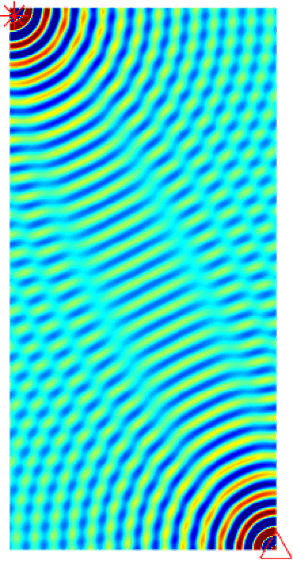## Numerical tests for seismic resolution

Seismic resolution and fidelity are the two important measures of the quality of the seismic record and the seismic images. Seismic resolution quantifies the...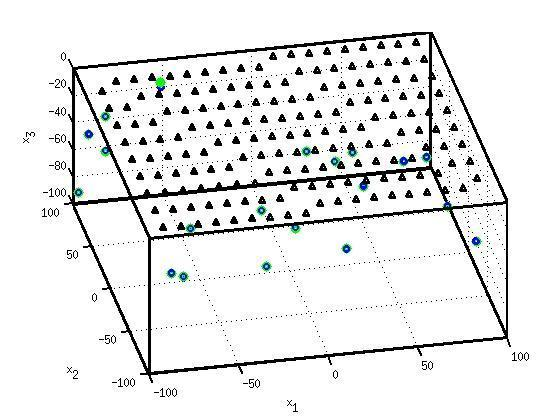## Locating earthquakes using geiger’s method

Simple earthquake location problem and its solution using Geiger’s method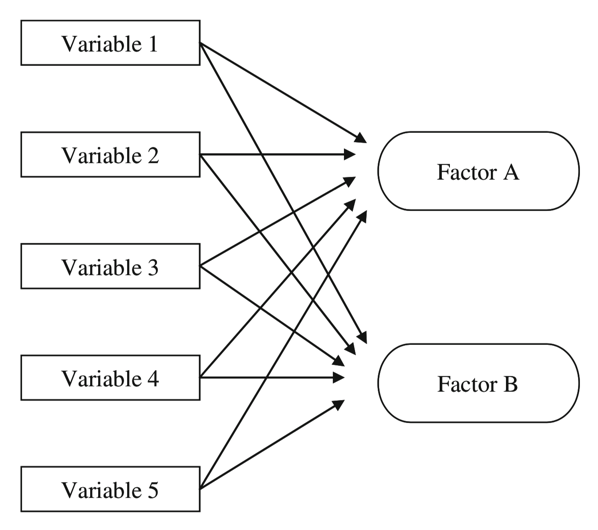## Exploratory factor analysis

Factor Analysis is an exploratory data analysis method used to search influential underlying factors or latent variables from a set of observed variables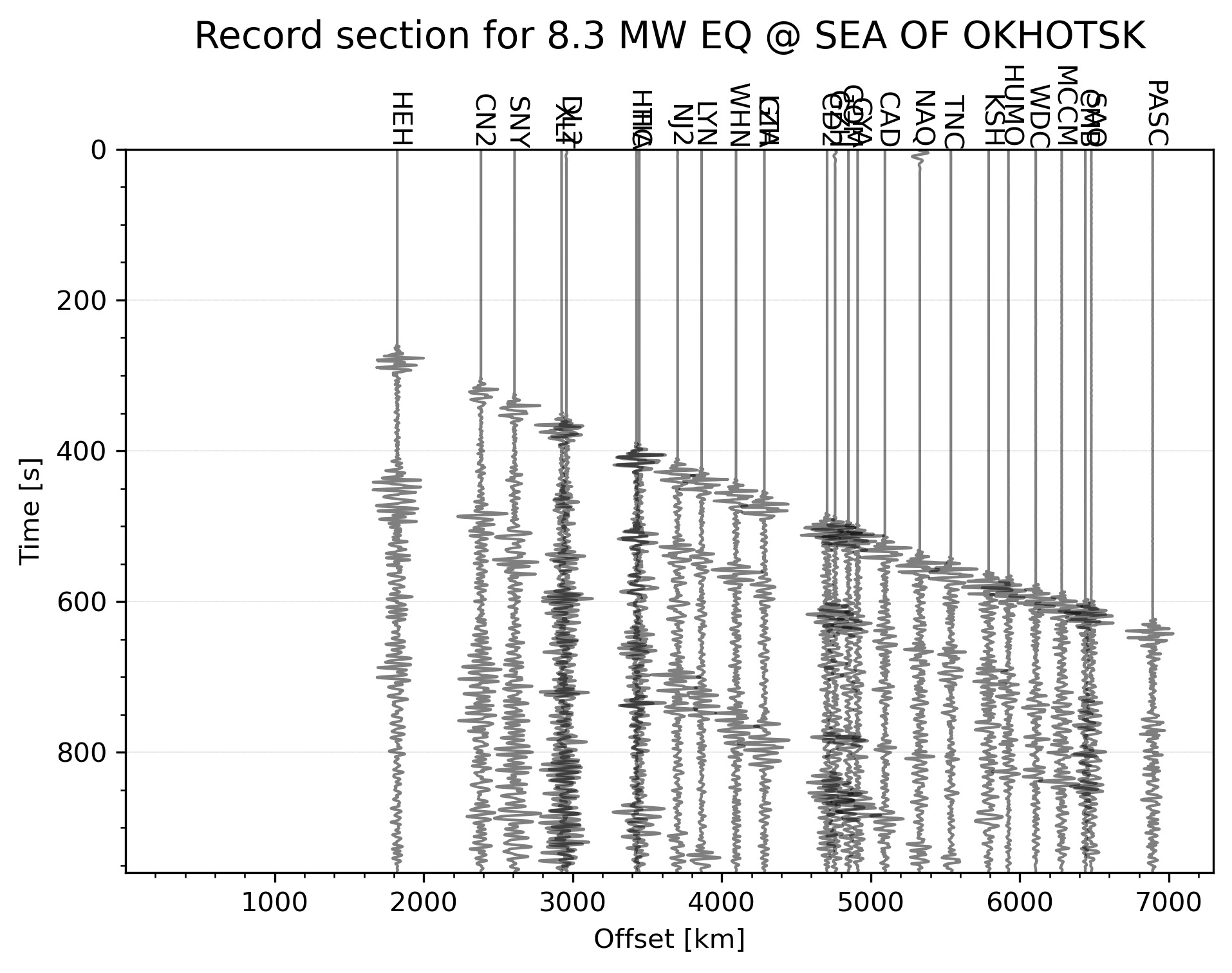## Automatically plotting record section for an earthquake in the given time range [python]

Python code to automatically plot the record section for the highest magnitude earthquake in the given time range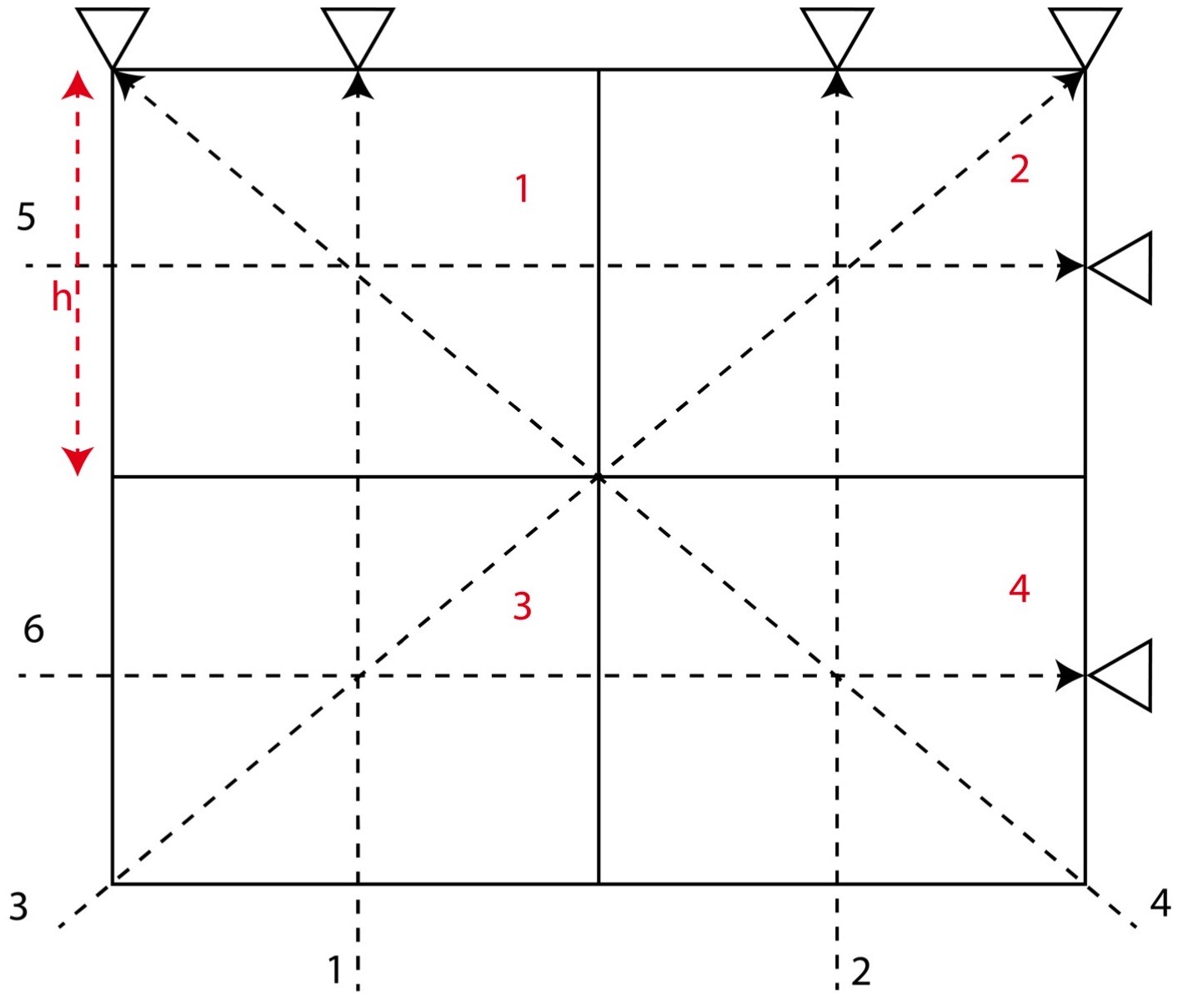## Numerical tests on travel time tomography [matlab]

Introduction to the concepts of tomography with equations and codes. Introduction to the concepts of overdetermined, underdetermined and mix-determined probl...

## Mapping Tutorials## Pygmt: high-resolution topographic map in python

A simple tutorial on how to plot high resolution topographic map using GMT tools in Python## Topographic map clipped by coastlines [python]

This post demonstrate how to use Python to set up clip topographic map based on coastlines.

## Seismology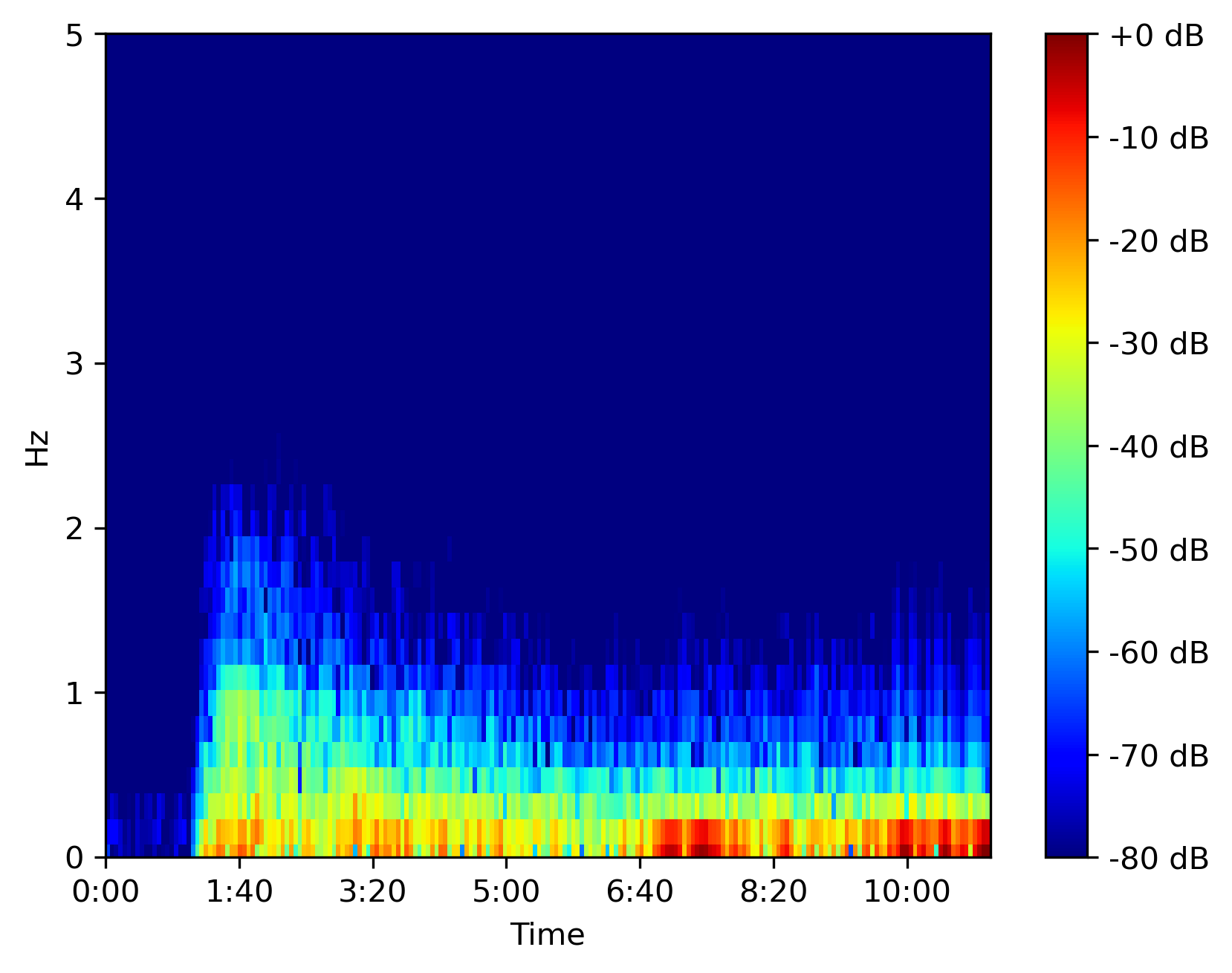## Efficiently compute spectrogram for large dataset in python

Librosa can efficiently compute the spectrogram for large time series data in seconds. We will use that to plot the spectrogram using matplotlib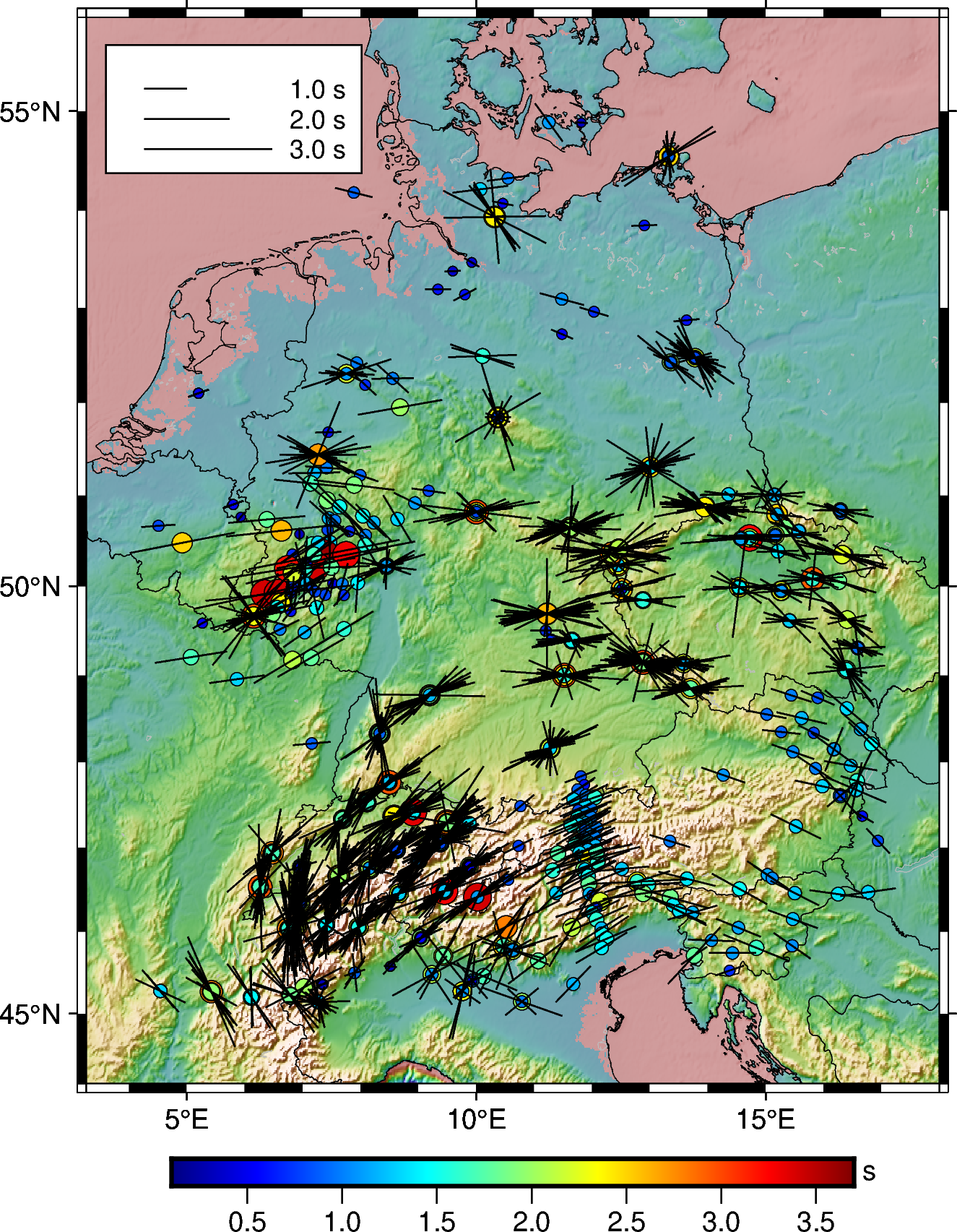## How to plot shear-wave splitting measurements using pygmt

How you can plot the shear-wave splitting measurements from splitting database using PyGMT.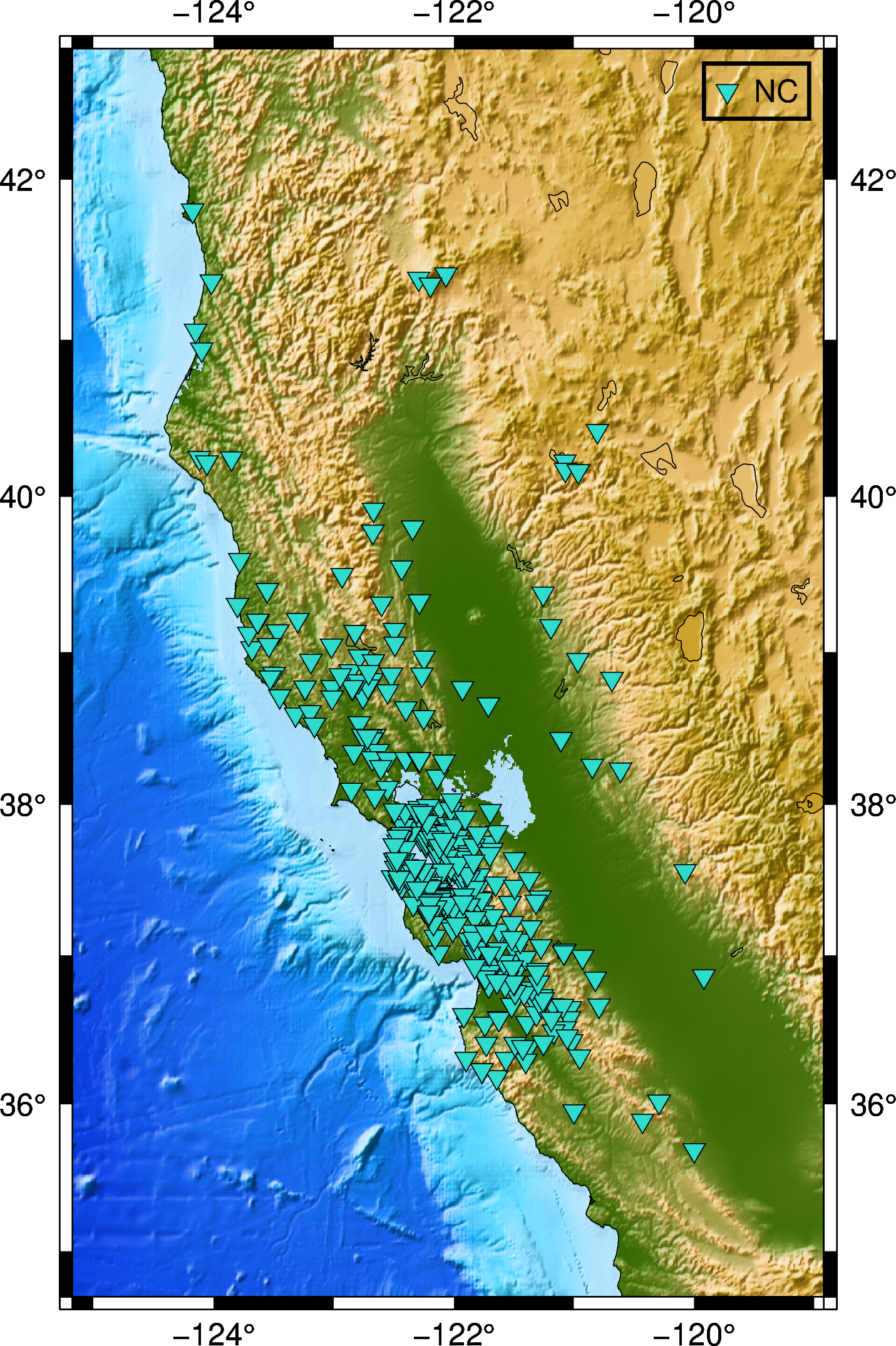## How to automatically enquire the availability of seismic data using obspy

In this post, we will see how can we retrive the available seismic waveforms information for a given network, station, channel and client in a given period o...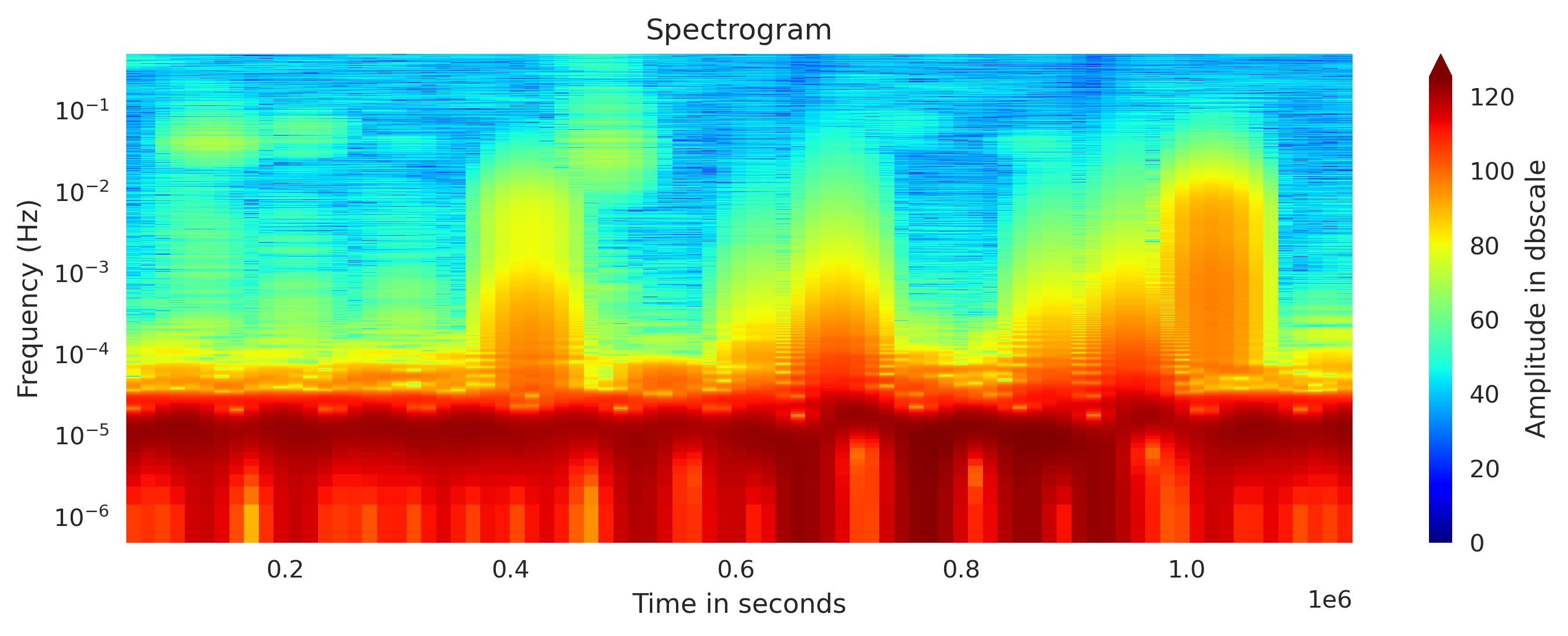## Concatenating daily seismic traces into one miniseed file (codes included)

I concatenate the daily seismic traces for 15 days into one miniseed file for further analysis. Then I obtained the spectrogram of the 15 days seismic data. ...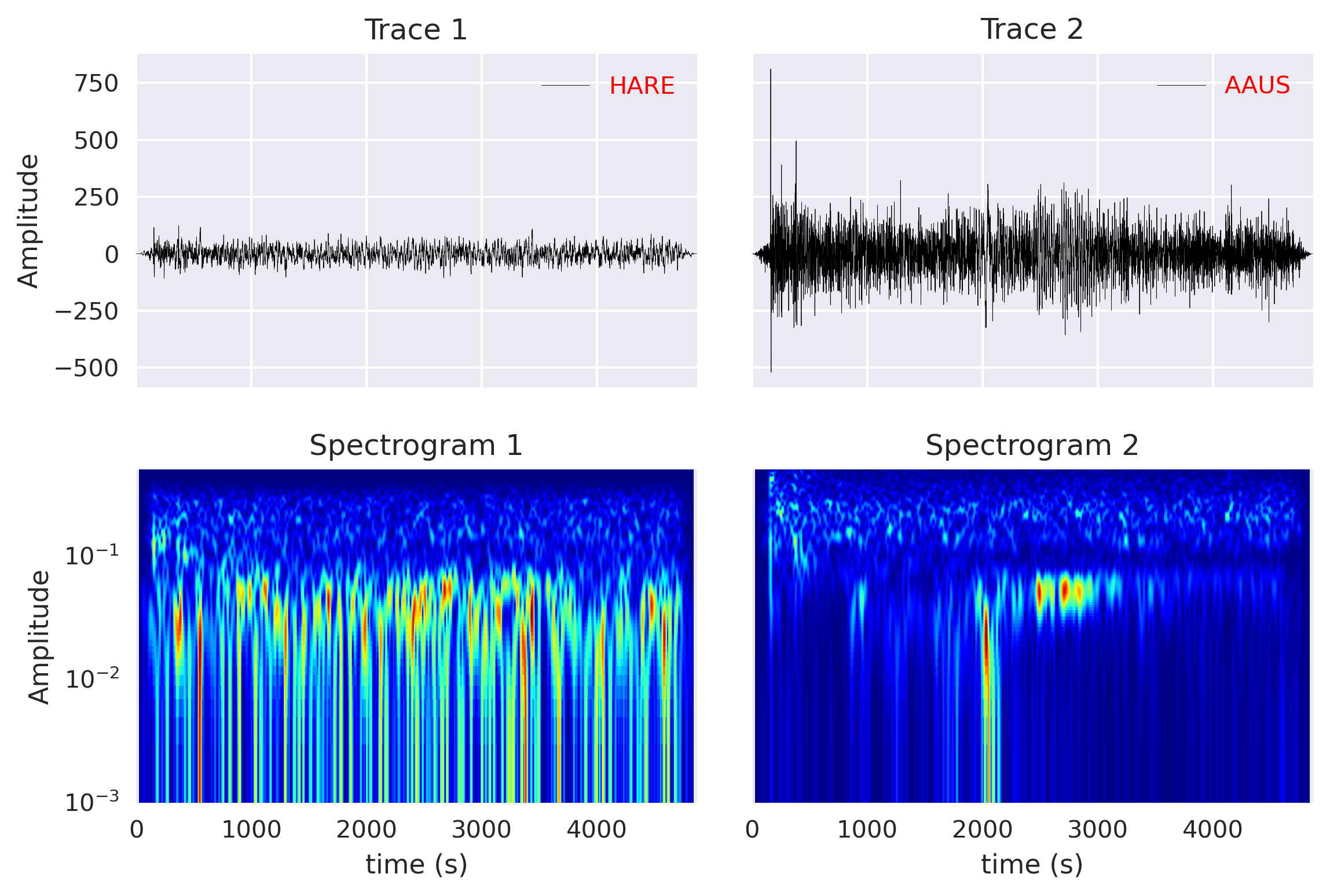## Computing cross-correlation and spectrogram of two seismic traces (codes included)

Read the seismic traces from the miniseed files and compute the cross-correlation and spectrogram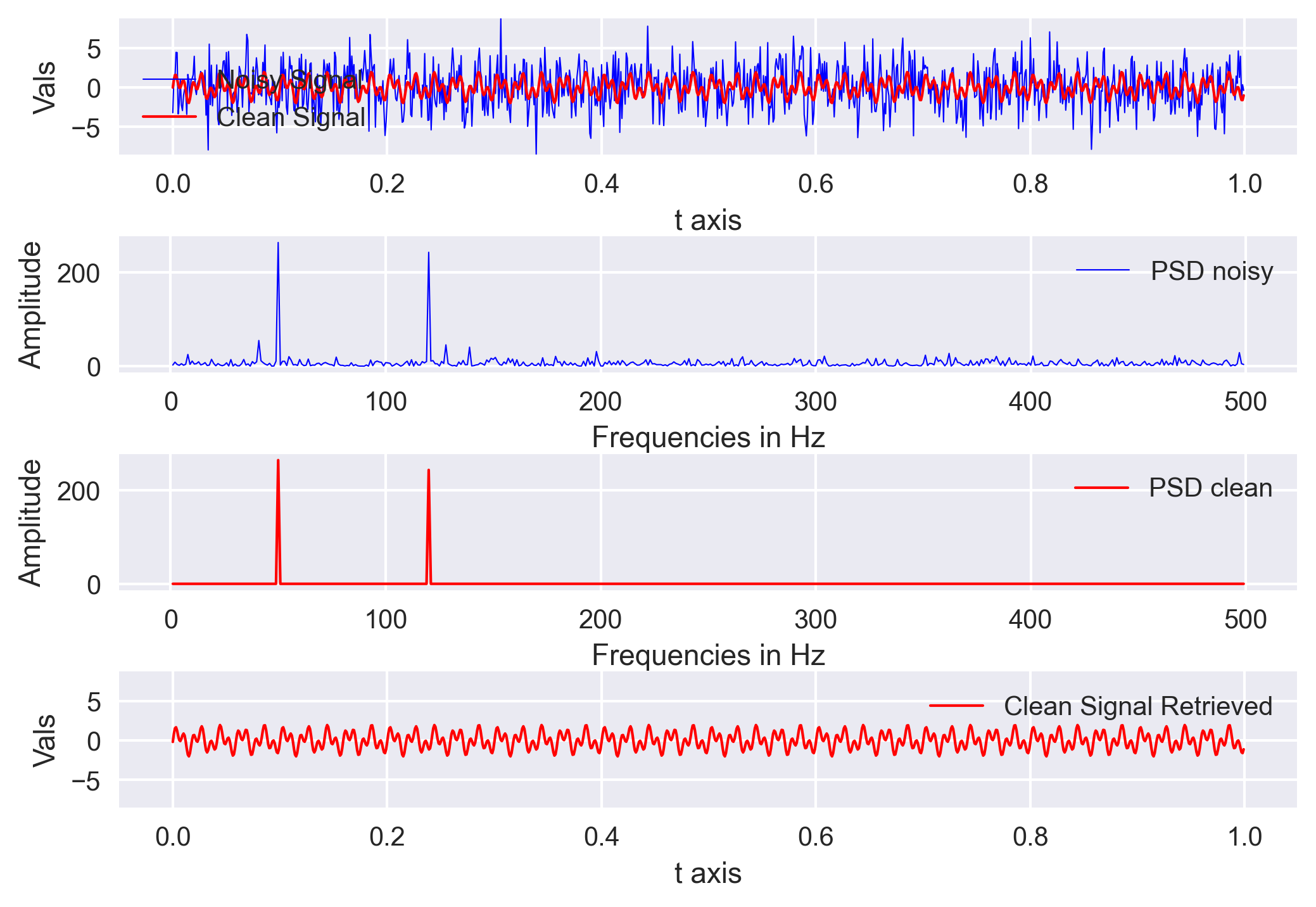## Signal denoising using fourier analysis in python (codes included)

We will learn the basics of Fourier analysis and implement it to remove noise from the synthetic and real signals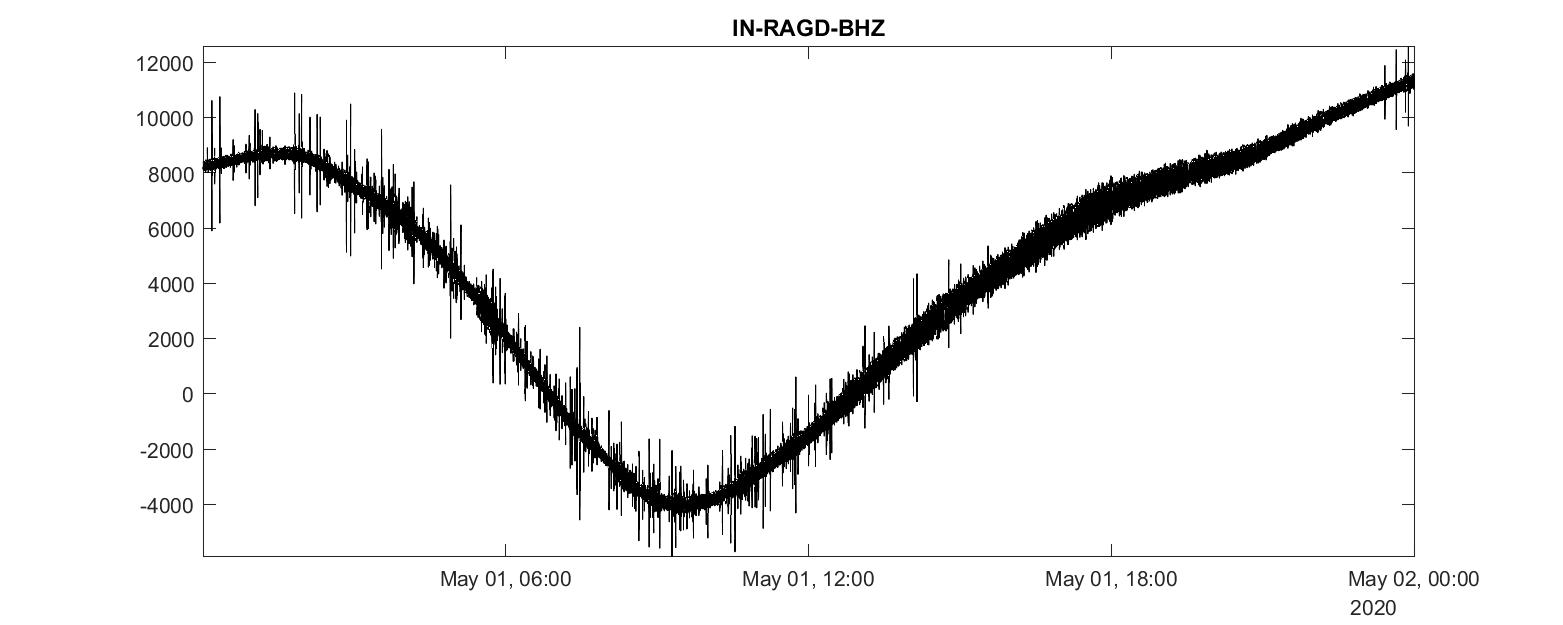## Analyzing miniseed seismic data in matlab (codes included)

We will learn how to convert a mseed data file into mat format and then read and analyze it using MATLAB## The easy way to compute and visualize the time & frequency domain correlation (codes included)

In geophysics, it is important to understand and identify the complex and unknown relationships between two time-series. Cross-correlation is an established ...## Plotting a record section using obspy (codes included)

Quickly plot record section of a stream using Obspy. I will introduce you how to make a stream from a set of SAC data, plot the record section and store it a...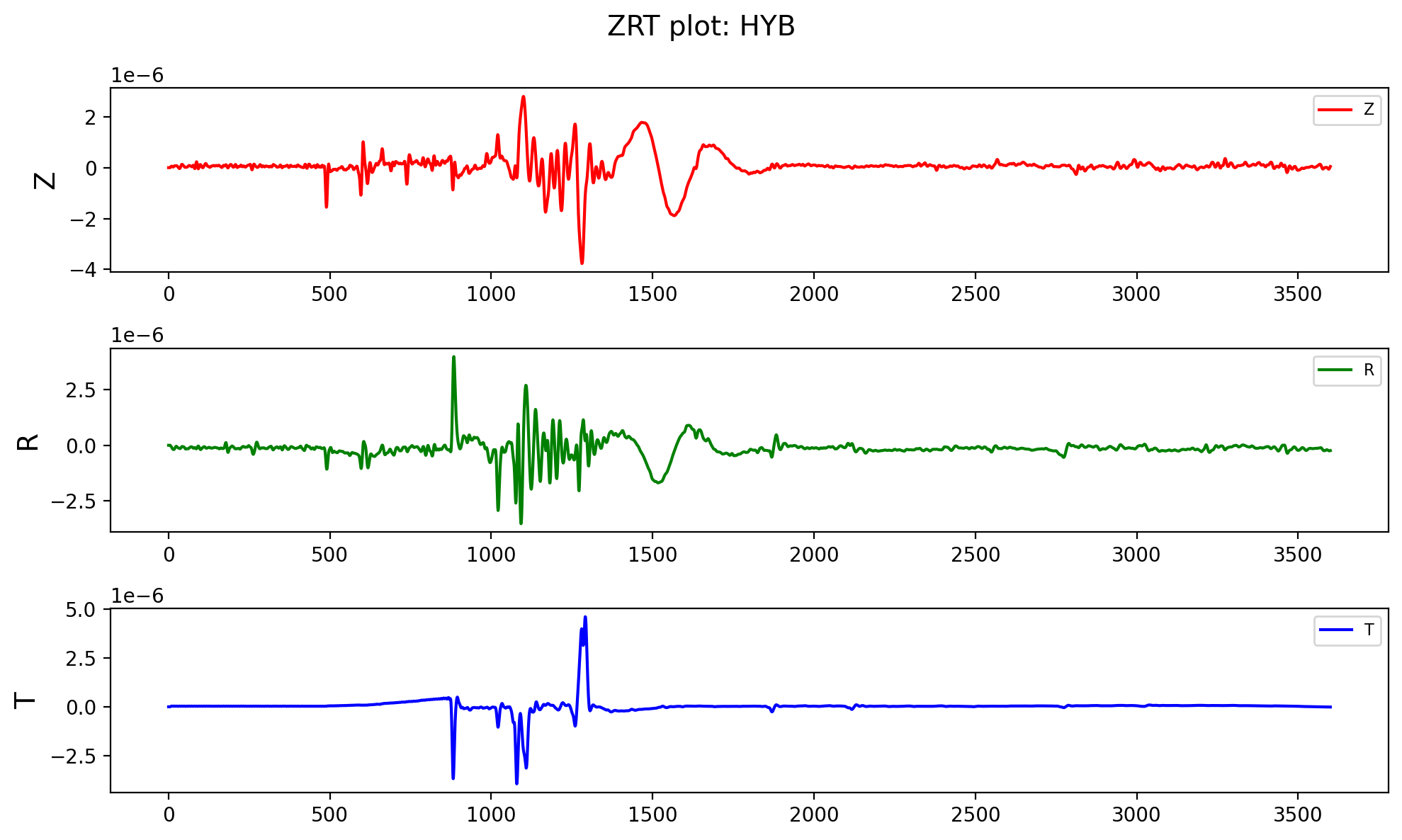## Write ascii data to mseed file using obspy (codes included)

In this post, I will read a ASCII file whose first few lines contains the header information and then the three-component data. I will read using the pandas ...## Numerical tests for seismic resolution (codes included)

Seismic resolution and fidelity are the two important measures of the quality of the seismic record and the seismic images. Seismic resolution quantifies the...## Locating earthquakes using geiger’s method (codes included)

Simple earthquake location problem and its solution using Geiger’s method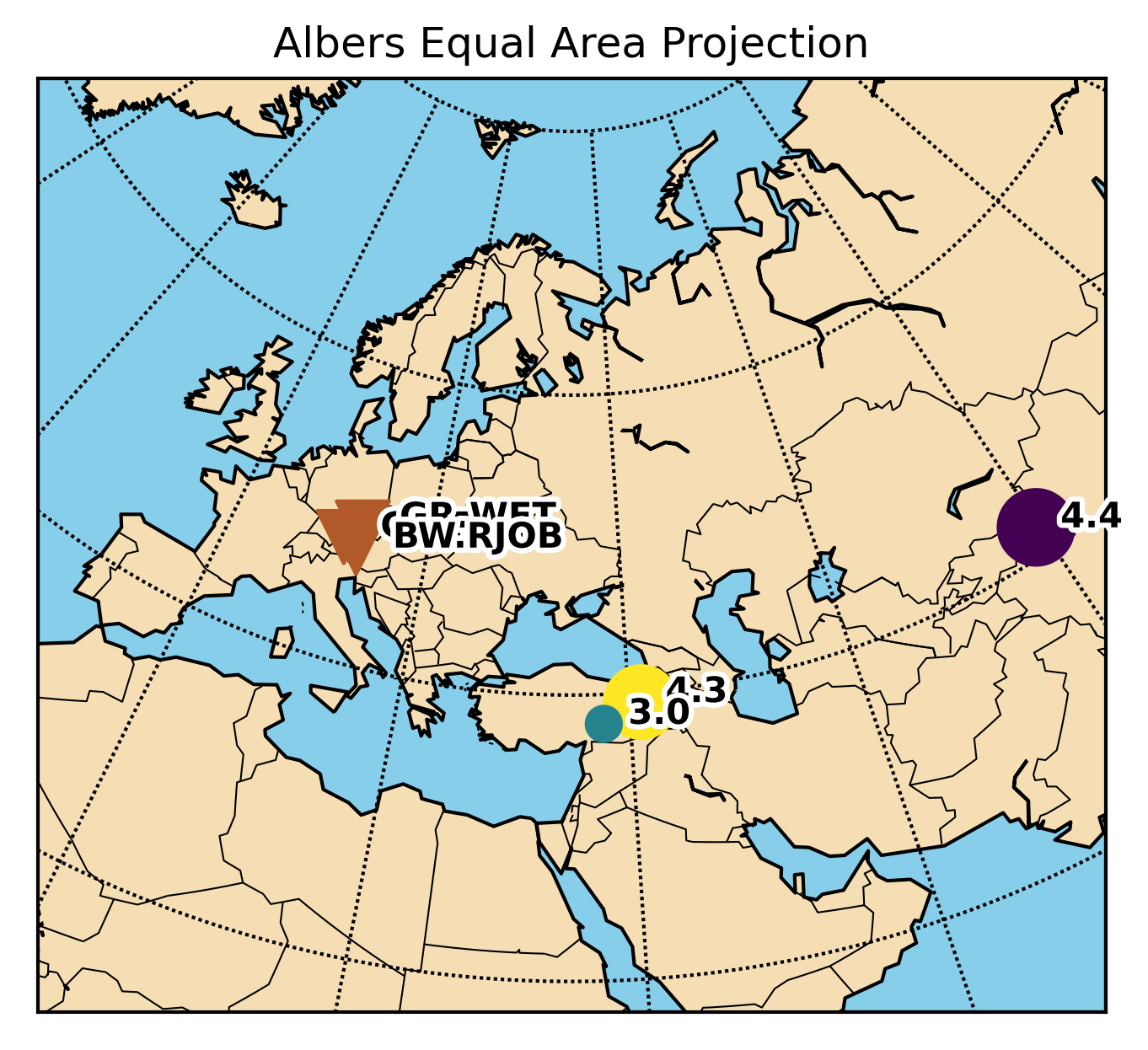## Working with obspy and basemap (codes included)

This post is aimed to resolve the issues regarding the conflicts of using obspy and basemap libraries together.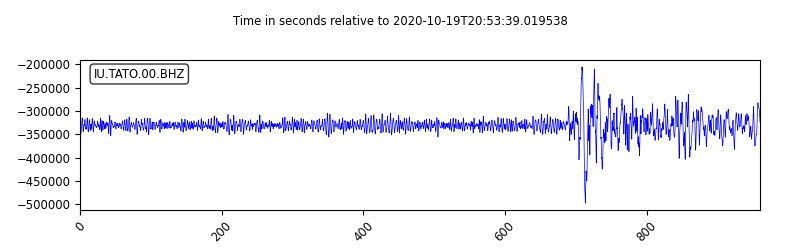Obspy is an open-source Python framework developed for the processing of seismological data. In this post, I will introduce how to use Obspy along with some ...## Pygmt: high-resolution topographic map in python (codes included)

A simple tutorial on how to plot high resolution topographic map using GMT tools in Python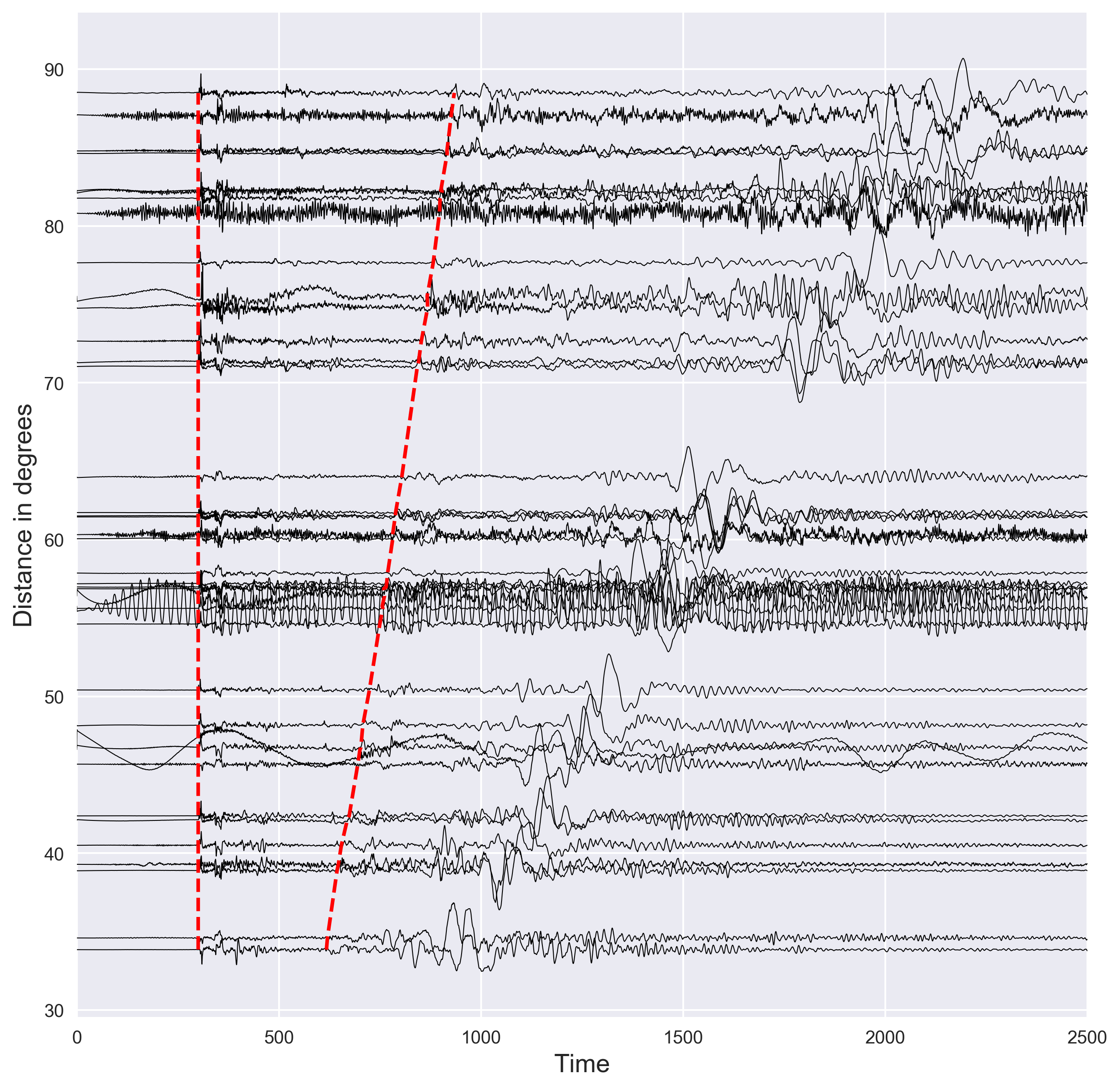## Plotting seismograms with increasing epicentral distance using python (codes included)

Short demostration of how to plot the distance vs seismic waveforms and mark the P and S arrival times using the IASP91 earth model. Codes are included.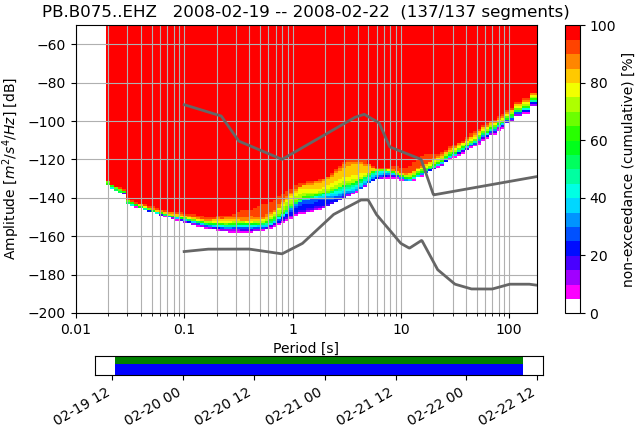## Visualizing power spectral density using obspy in python (codes included)

Short demonstration of the ppsd class defined in Obspy using 3 days of data for station PB-B075## Automatically plotting record section for an earthquake in the given time range in python (codes included)

Python code to automatically plot the record section for the highest magnitude earthquake in the given time range

## Numerical methods for scientific computation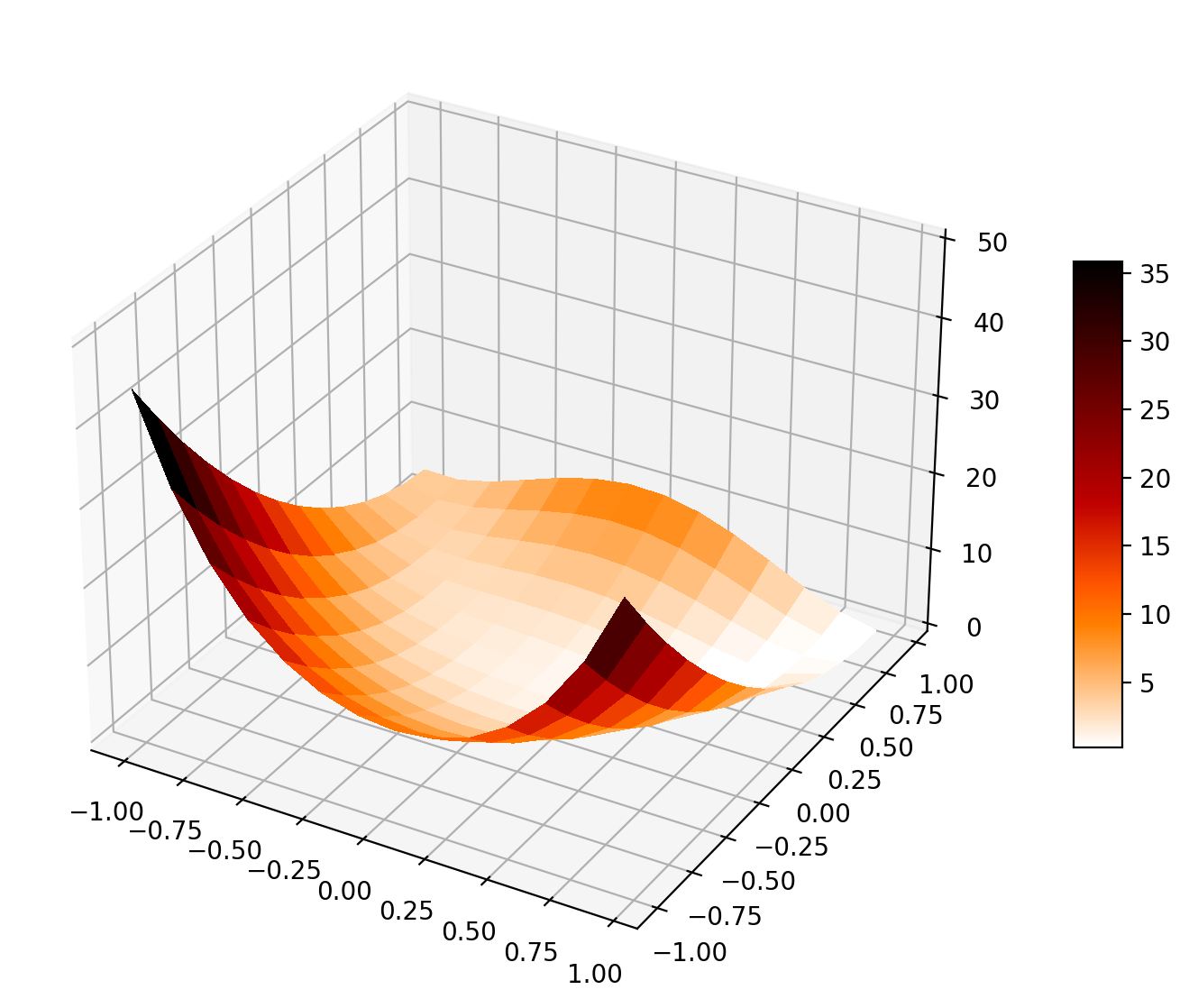## Numerical optimization based on the l-bfgs method

We will inspect the L-BFGS optimization method using one example and compare its performance with the gradient descent method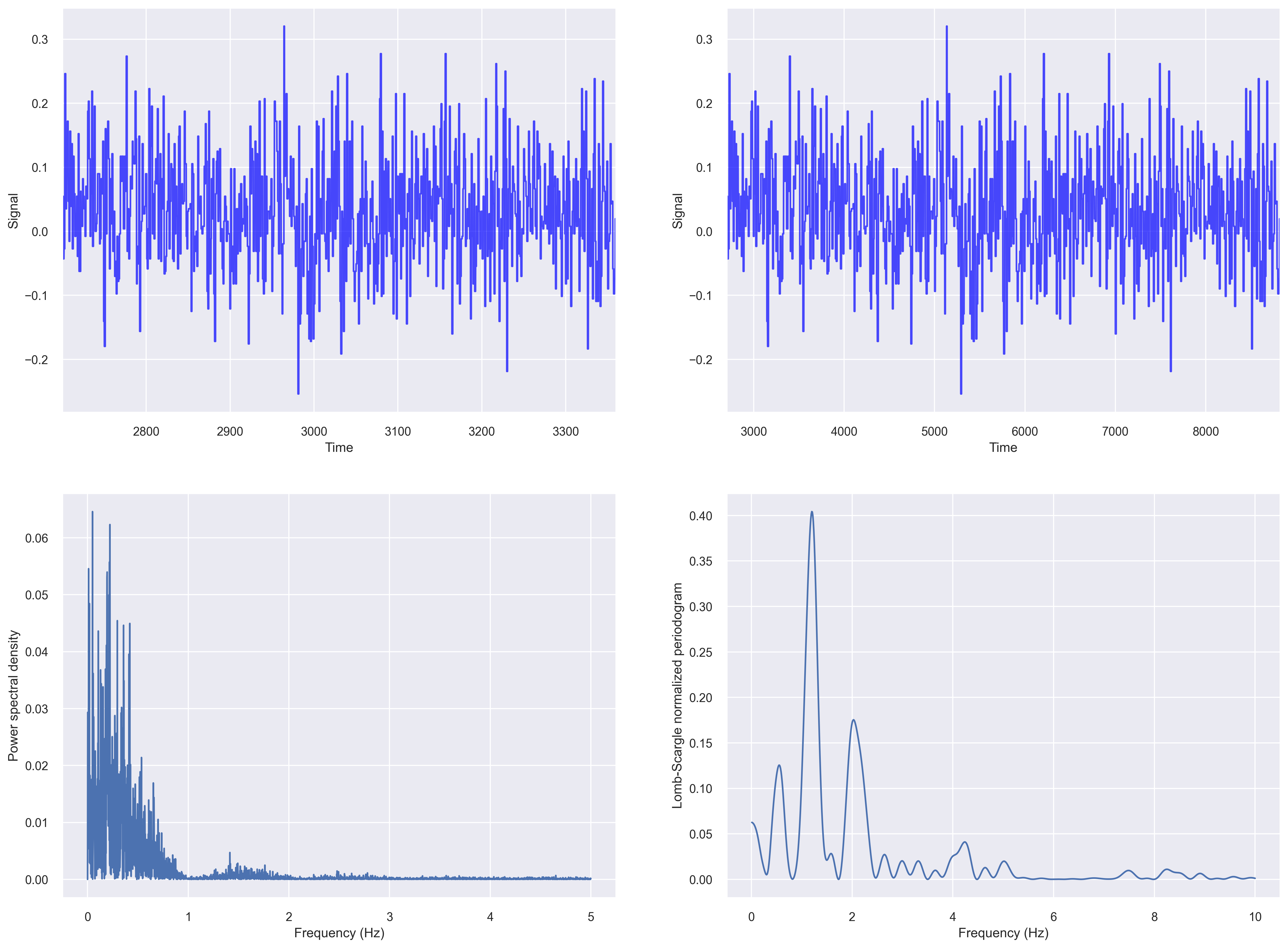## How to deal with irregularly sampled time series data

While analyzing time series data, we often come across data that is non-uniformly sampled, i.e., they have non-equidistant time-steps. Infact, most of the re...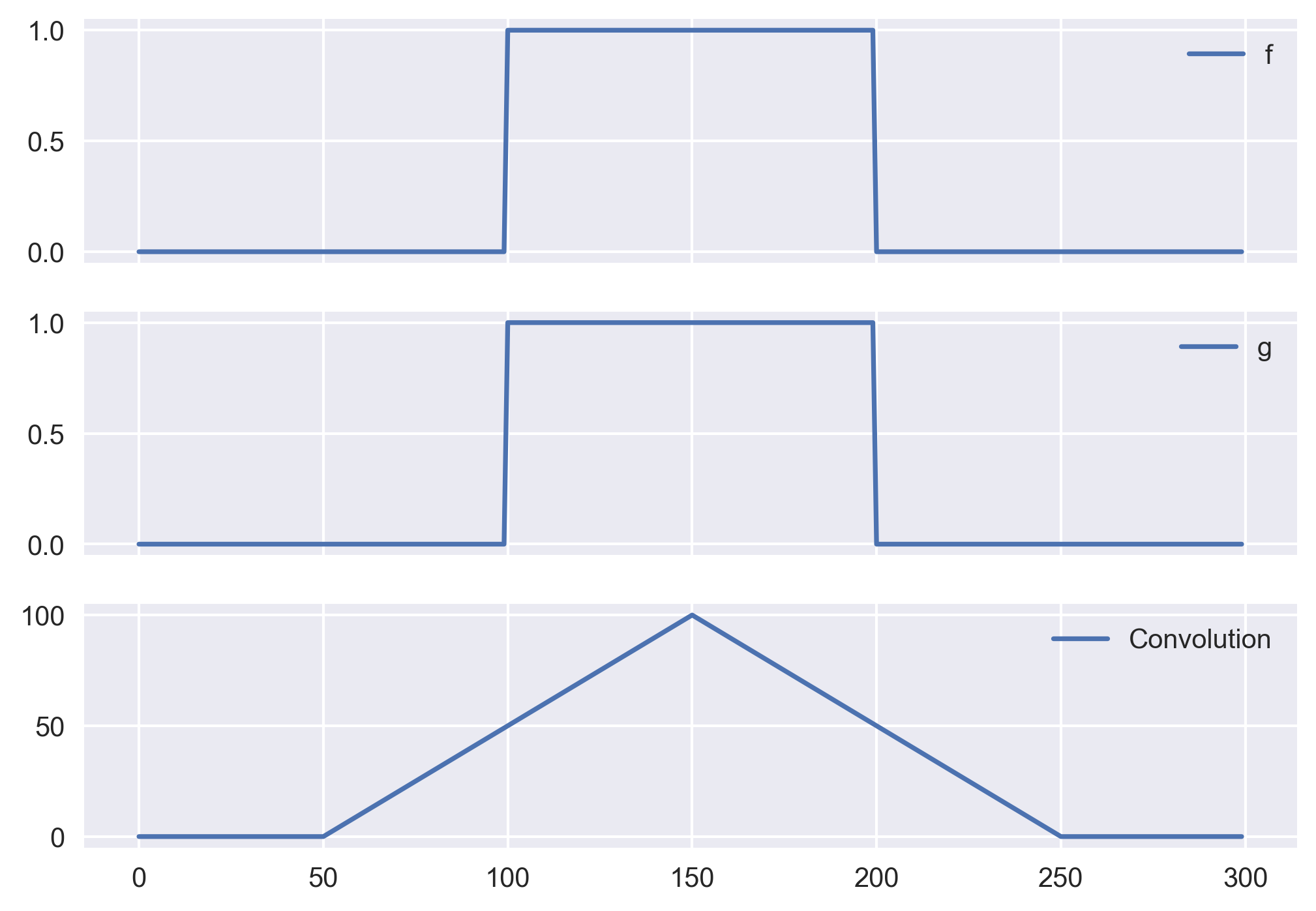## Convolution of waveforms using python compared to matlab

We will see compare the convolution functions in Python (Numpy) with the conv function in MATLAB. If you have tried them both then you would know that its no...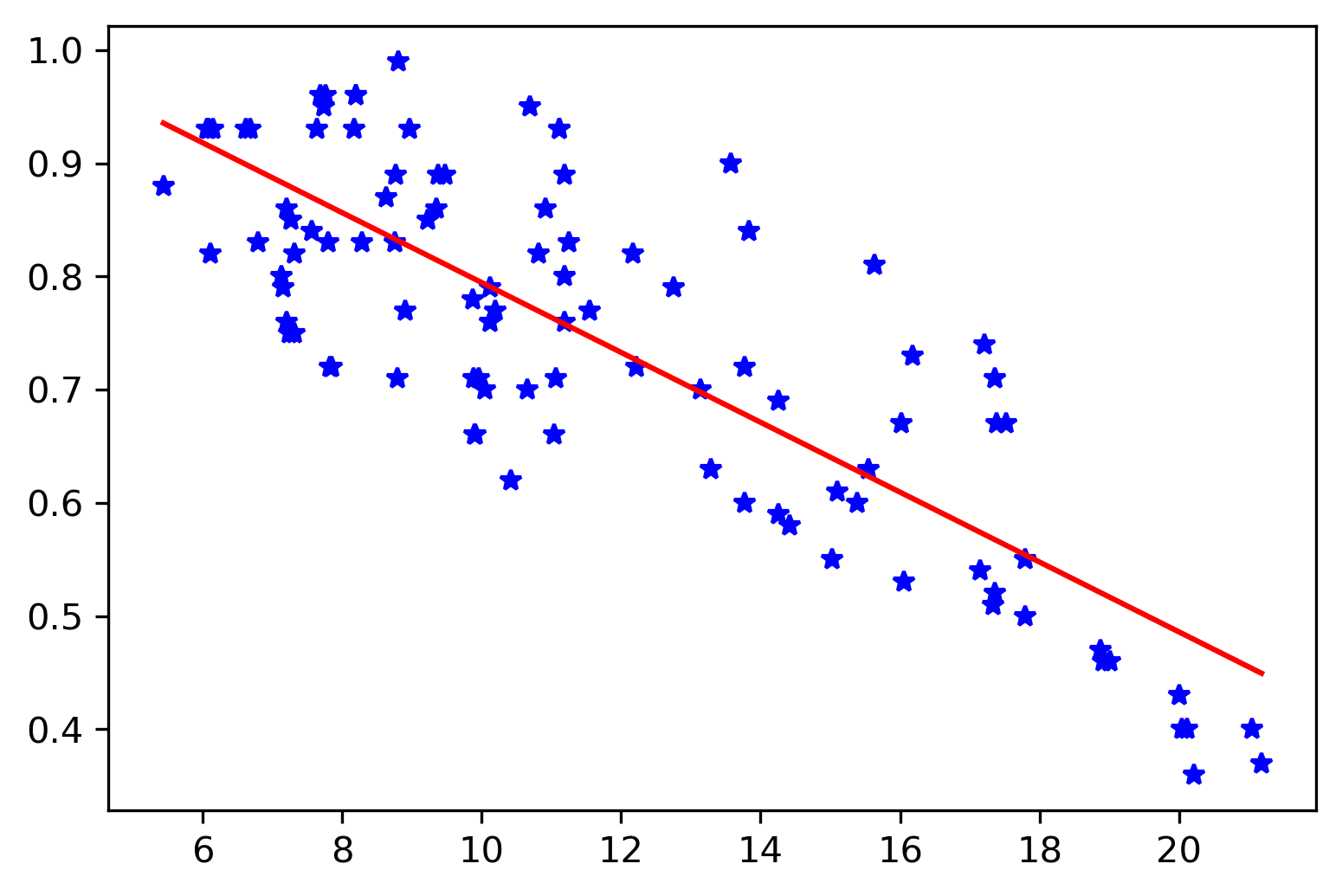## Maximum likelihood estimation for the regression parameters

We will learn the basics of the maximum likelihood method, and then apply it on a regression problem. We will also compare it with the least-squares estimati...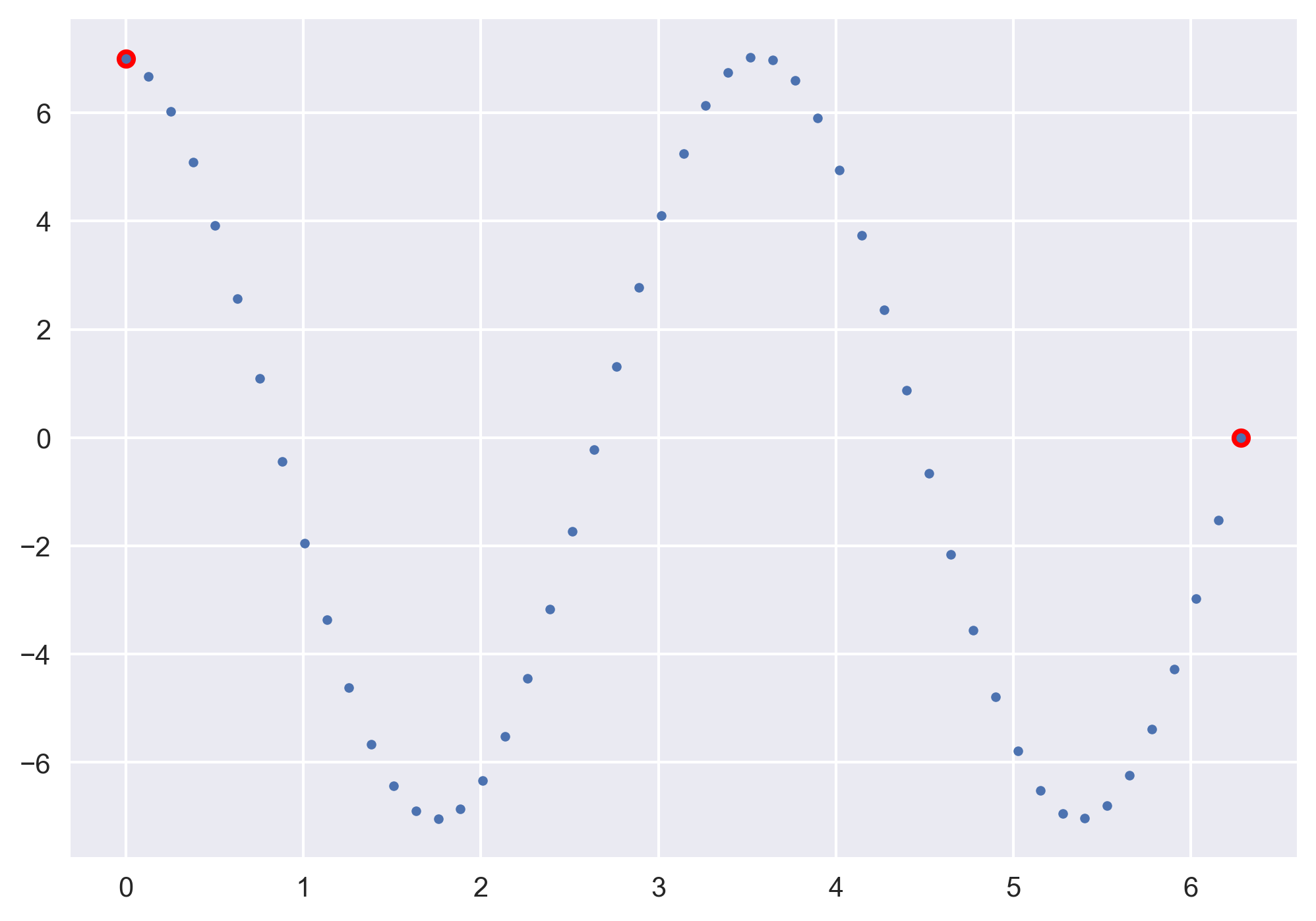## Solving boundary value problems using the shooting method

The boundary value problems require information at the present time and a future time. We will see how we can use shooting method to solve problems where we ...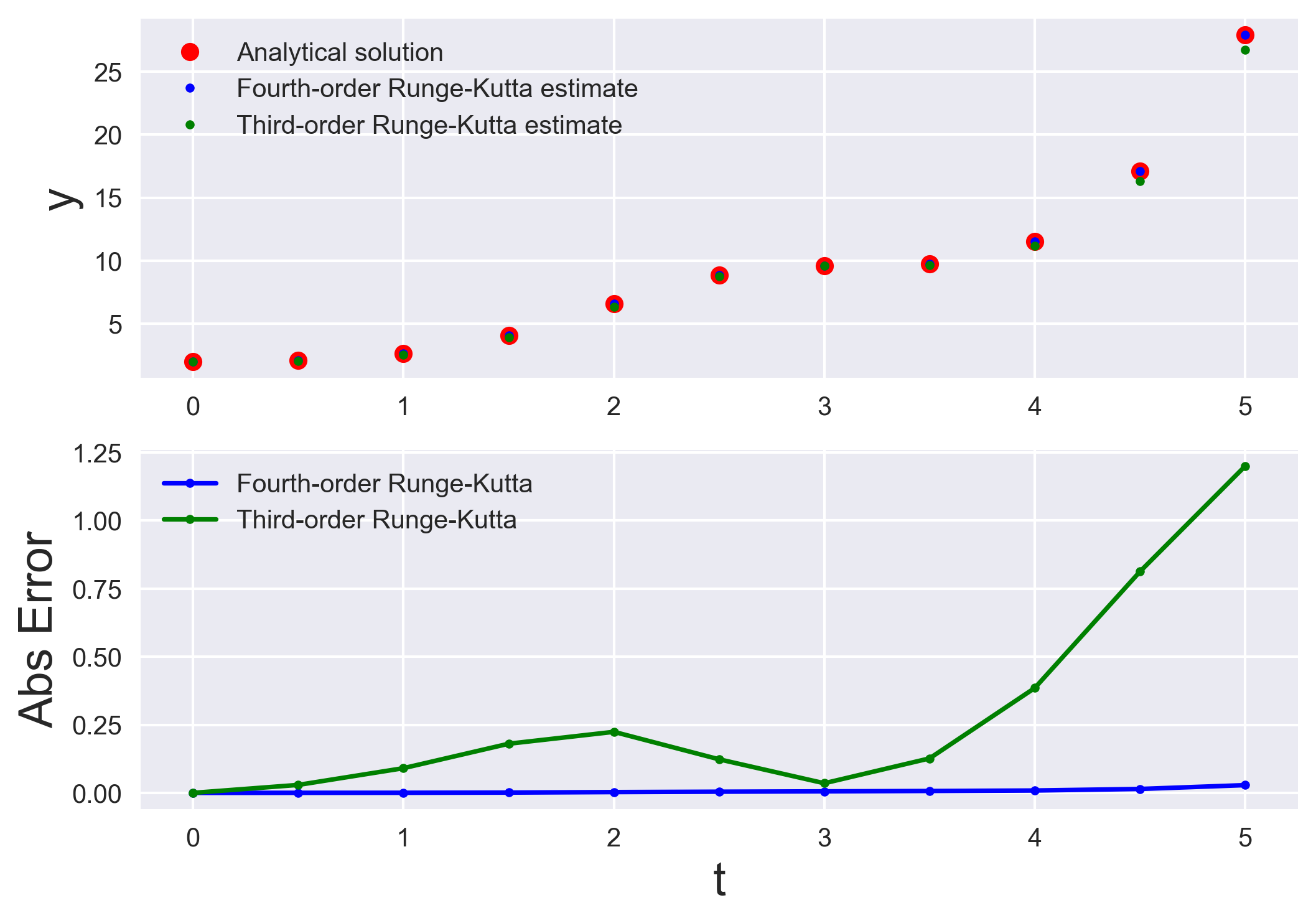## Numerically solving initial value problems using the runge-kutta method

Runge-Kutta methods are most popular method to solve ordinary differential equations (ODEs) with a better approximation than the Euler method. We compare the...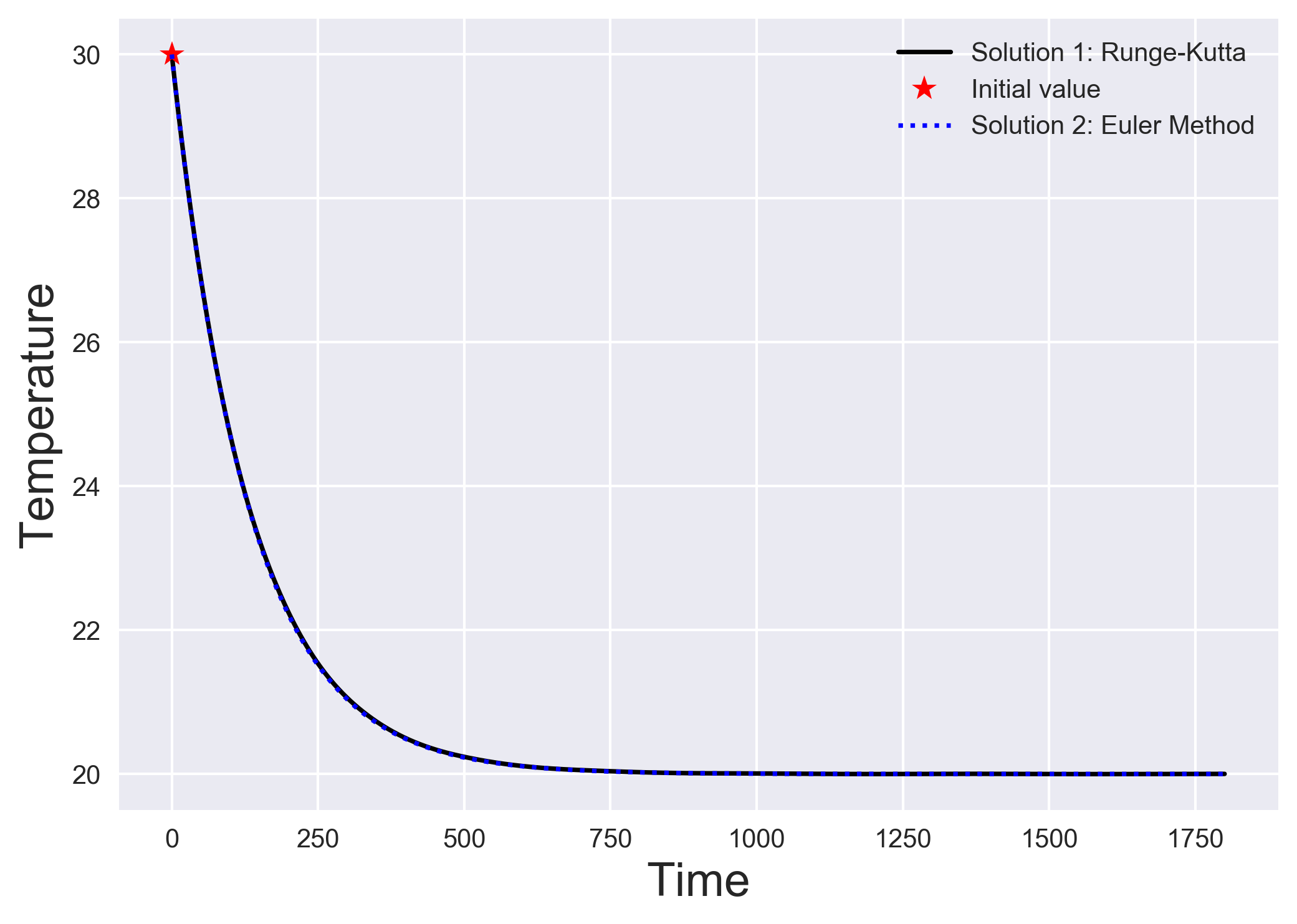## Numerically solving initial value problems using the euler method

The simplest algorithm to solve a system of differential equations is the Euler method. We understand the Euler method by looking into a simple heat transfer...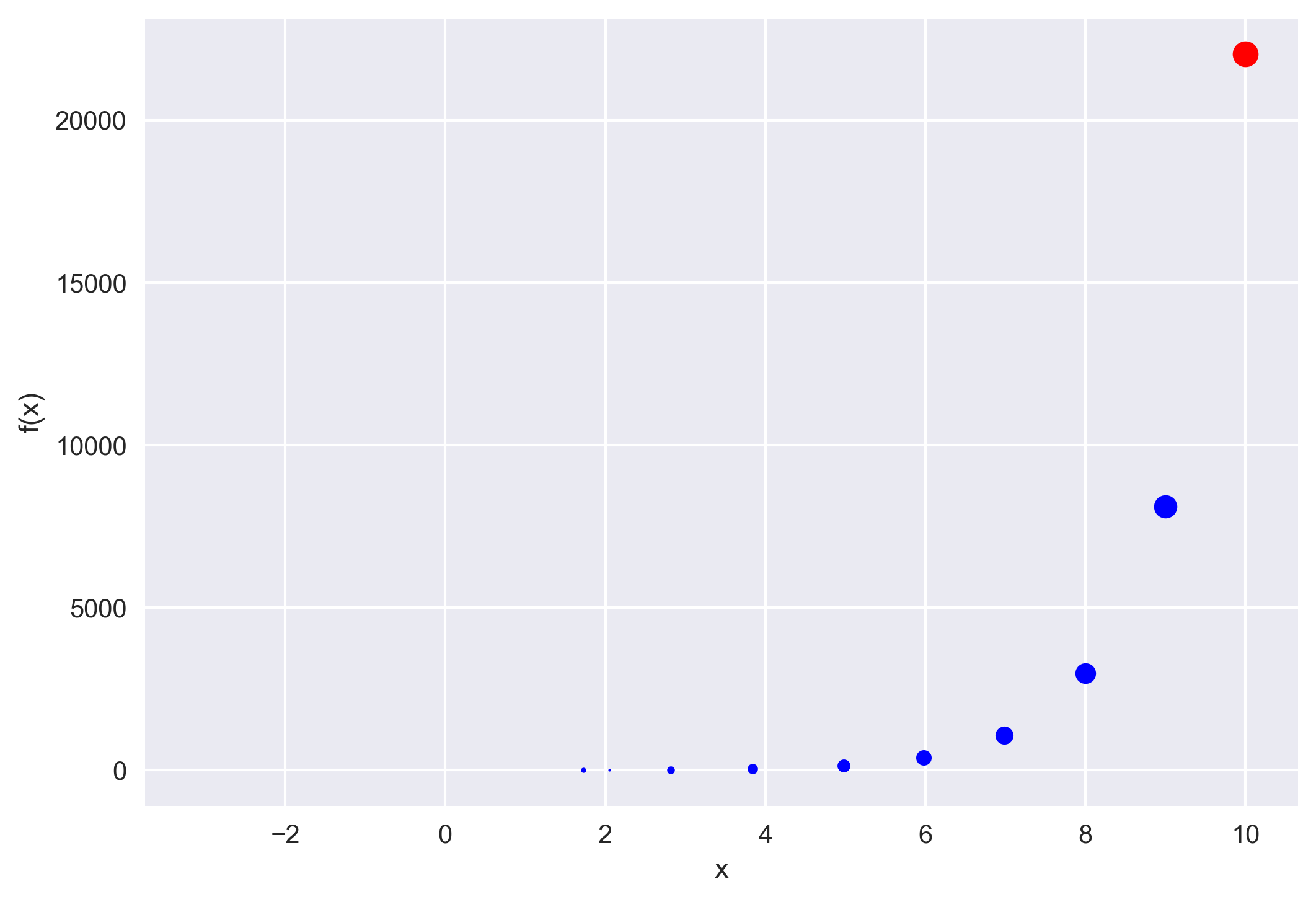## How to implement the iterative newton–raphson method to find roots of a function in python

The Newton–Raphson method (commonly known as Newton’s method) is developed for finding roots of a given function or polynomial iteratively. We show two examp...## Empirical orthogonal function analysis to inspect the spatial coherency in the geospatial data (codes included)

Empirical Orthogonal Functions analysis decomposes the continuous space-time field into a set of orthogonal spatial patterns along with a set of associated u...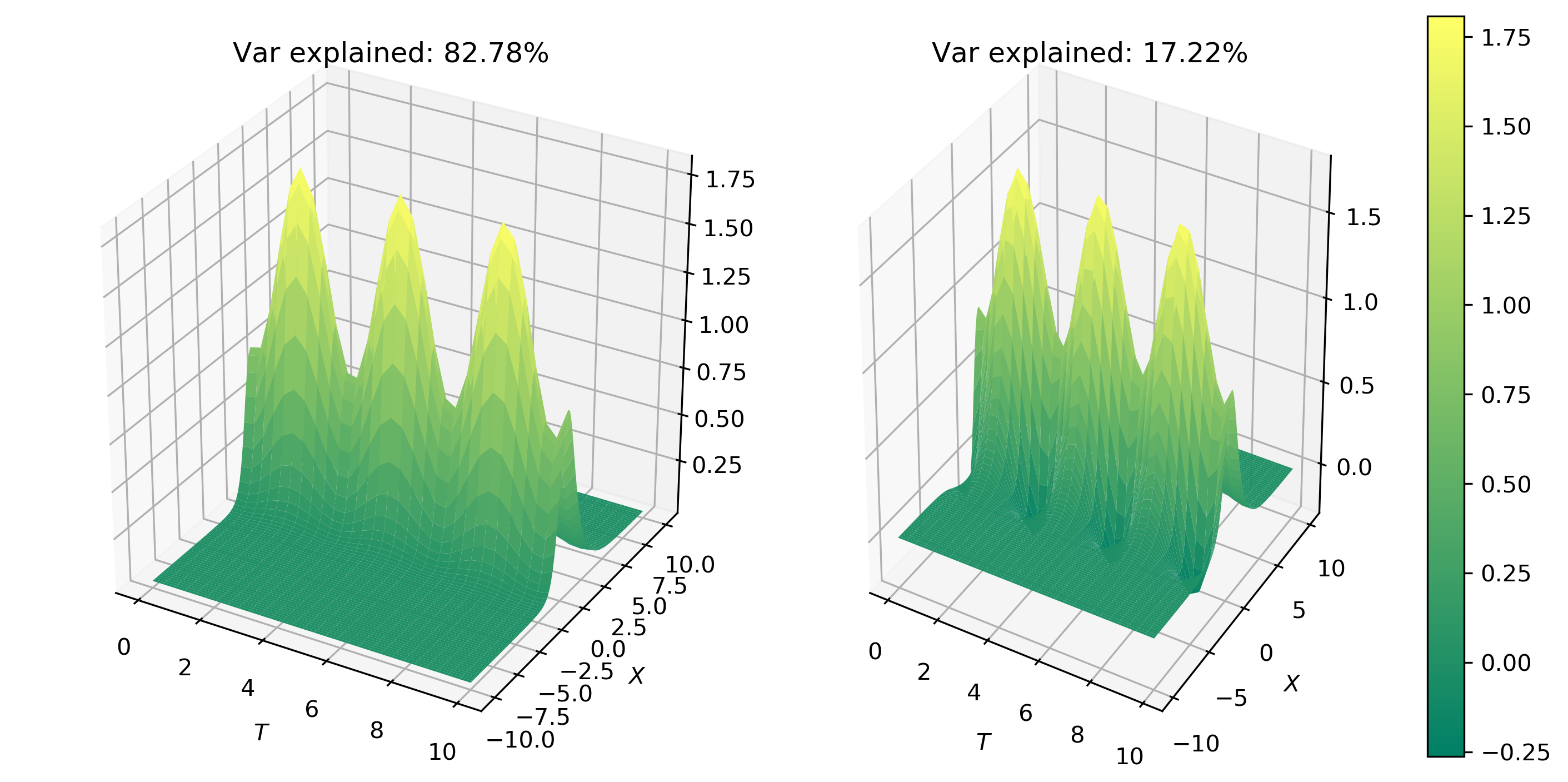## Principal component analysis to decompose signals and reduce dimensionality (codes included)

We will learn the basics of Fourier analysis and implement it to remove noise from the synthetic and real signals## Genetic algorithm: a highly robust inversion scheme for geophysical applications (codes included)

An introduction to the basics of genetic algorithm along with a simple numerical example and solution of an earthquake location problem## Monte carlo methods and earthquake location problem (codes included)

The common geophysical problems most often have multimodal objective function with many possible minima. In this post, we will look into the Monte Carlo meth...## Least-squares method in geosciences (codes included)

Least-squares method is a popular approach in geophysical inversion to estimate the parameters of a postulated Earth model from given observations. This meth...## Numerical tests for seismic resolution (codes included)

Seismic resolution and fidelity are the two important measures of the quality of the seismic record and the seismic images. Seismic resolution quantifies the...## Exploratory factor analysis (codes included)

Factor Analysis is an exploratory data analysis method used to search influential underlying factors or latent variables from a set of observed variables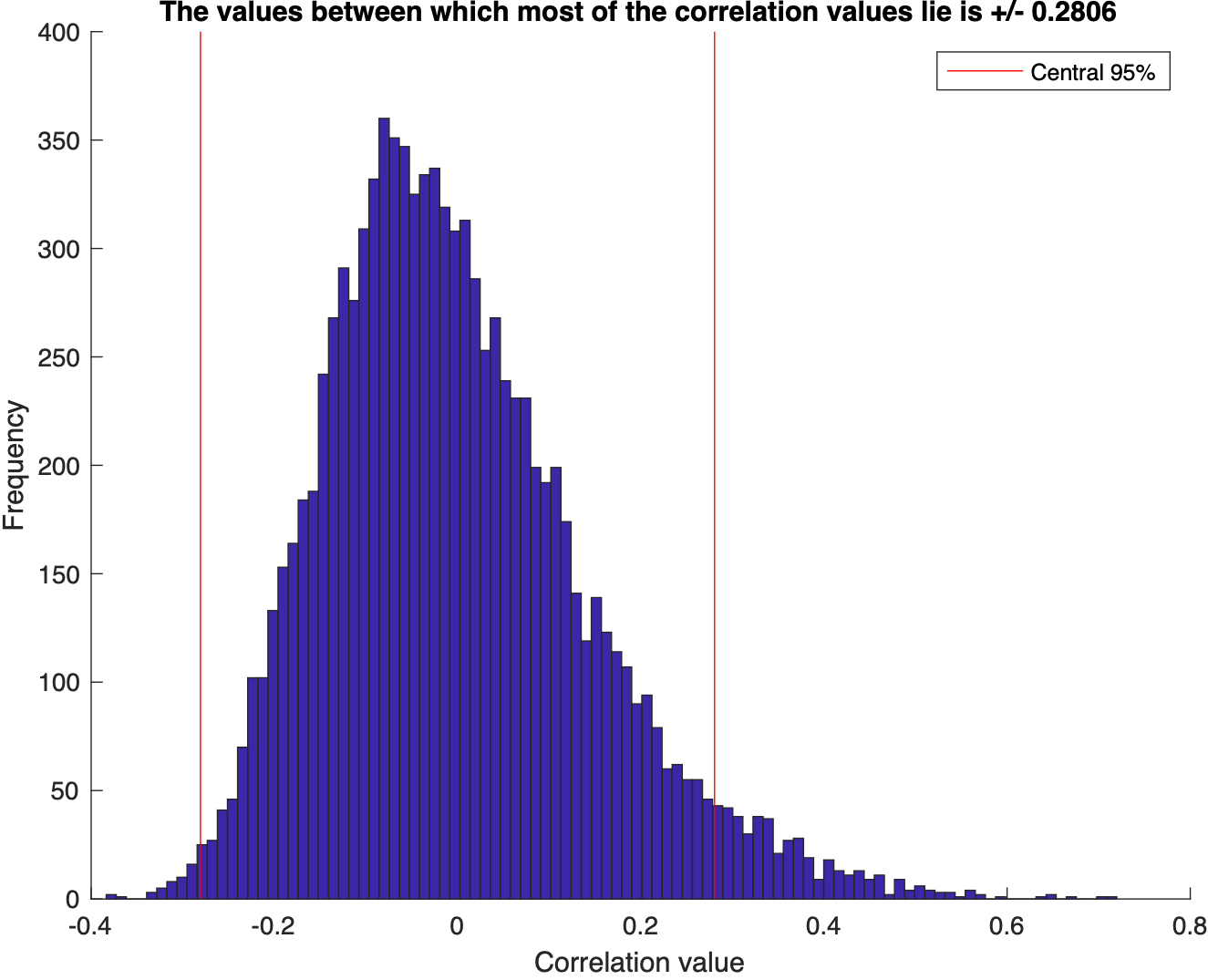## Monte carlo simulation to test for the correlation between two dataset in matlab (codes included)